SLVSBH3B June   2016  – April 2017

PRODUCTION DATA.

1. Features
2. Applications
3. Description
4. Revision History
5. Device Comparison Table
6. Pin Configuration and Functions
7. Specifications
8. Parameter Measurement Information
9. Detailed Description
1. 9.1 Overview
2. 9.2 Functional Block Diagram
3. 9.3 Feature Description
4. 9.4 Device Functional Modes
10. 10Application and Implementation
1. 10.1 Application Information
2. 10.2 Typical Applications
1. 10.2.1 Typical Application with Adjustable Output Voltage
2. 10.2.2 Typical Application using VSEL and FB2
3. 10.3 System Examples
11. 11Power Supply Recommendations
12. 12Layout
13. 13Device and Documentation Support
14. 14Mechanical, Packaging, and Orderable Information

• RGX|11

## 10 Application and Implementation

NOTE

Information in the following applications sections is not part of the TI component specification, and TI does not warrant its accuracy or completeness. TI’s customers are responsible for determining suitability of components for their purposes. Customers should validate and test their design implementation to confirm system functionality.

### 10.1 Application Information

#### 10.1.1 Programming the Output Voltage

The output voltage of the TPS62135x is adjustable. It can be programmed for output voltages from 0.8 V to 12 V, using a resistor divider from VOUT to GND. The voltage at the FB pin is regulated to 700 mV. The value of the output voltage is set by the selection of the resistor divider from Equation 8. It is recommended to choose resistor values which allow a current of at least 2 uA, meaning the value of R2 should not exceed 400 kΩ. Lower resistor values are recommended for highest accuracy and most robust design.

Equation 8.#### 10.1.2 External Component Selection

The external components have to fulfill the needs of the application, but also the stability criteria of the device´s control loop. The TPS62135x is optimized to work within a range of external components. The LC output filters inductance and capacitance have to be considered together, creating a double pole, responsible for the corner frequency of the converter (see Output Filter and Loop Stability). Table 2 can be used to simplify the output filter component selection.

### Table 2. Recommended LC Output Filter Combinations(1)

4.7 µF 10 µF 22 µF 47 µF 100 µF 200 µF ≥400 µF
0.68 µH
1 µH (2) (3)
1.5 µH (3)
2.2 µH (3)
3.3 µH
The values in the table are nominal values.
This LC combination is the standard value and recommended for most applications.
Output capacitance needs to have a ESR of ≥ 10 mΩ for stable operation, see also Powering Multiple Loads.

#### 10.1.3 Inductor Selection

The TPS62135x is designed for a nominal 1-µH inductor. Larger values can be used to achieve a lower inductor current ripple but they may have a negative impact on efficiency and transient response. Smaller values than 1µH will cause a larger inductor current ripple which causes larger negative inductor current in forced PWM mode at low or no output current. Therefore they are not recommended at large voltages across the inductor as it is the case for high input voltages and low output voltages. With low output current in forced PWM mode this causes a larger negative inductor current peak which may exceed the negative current limit. At low or no output current and small inductor values the output voltage can therefore not be regulated any more. More detailed information on further LC combinations can be found in SLVA463.

The inductor selection is affected by several effects like inductor ripple current, output ripple voltage, PWM-to-PFM transition point and efficiency. In addition, the inductor selected has to be rated for appropriate saturation current and DC resistance (DCR). Equation 9 calculates the maximum inductor current.

Equation 9.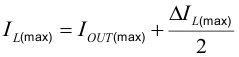Equation 10.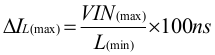where:

IL(max) is the maximum inductor current

ΔIL is the Peak to Peak Inductor Ripple Current

L(min) is the minimum effective inductor value.

Calculating the maximum inductor current using the actual operating conditions gives the minimum saturation current of the inductor needed. A margin of about 20% is recommended to add. A larger inductor value is also useful to get lower ripple current, but increases the transient response time and size as well. The following inductors have been used with the TPS62135x and are recommended for use:

### Table 3. List of Inductors

TYPE INDUCTANCE [µH] CURRENT [A](1) DIMENSIONS [LxBxH] mm MANUFACTURER
XFL4020-102ME 1.0 µH, ±20% 5.4 4 x 4 x 2.1 Coilcraft
XAL4020-102ME 1.0 µH, ±20% 8.7 4 x 4 x 2.1 Coilcraft
XAL4020-152ME 1.5 µH, ±20% 7.1 4 x 4 x 2.1 Coilcraft
XFL4030-102ME 1.0 µH, ±20% 4.1 4 x 4 x 3.1 Coilcraft
1277AS-H-1R0M (DFE322512C) 1.0 µH, ±20% 3.1(2) 3.2 x 2.5 x 1.2 Murata
Lower of IRMS at 40°C rise or ISAT at 30% drop.
For smallest size solutions that in average do not require the full output current TPS62135x can provide.

The inductor value also determines the load current at which Power Save Mode is entered:

Equation 11.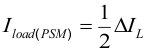#### 10.1.4.1 Output Capacitor

The recommended value for the output capacitor is 22 µF. The architecture of the TPS62135x allows the use of tiny ceramic output capacitors with low equivalent series resistance (ESR). These capacitors provide low output voltage ripple and are recommended. To keep its low resistance up to high frequencies and to get narrow capacitance variation with temperature, it is recommended to use X7R or X5R dielectric. Using a higher value has advantages like smaller voltage ripple and a tighter DC output accuracy in Power Save Mode (see SLVA463).

In Power Save Mode, the output voltage ripple depends on the output capacitance, its ESR, ESL and the peak inductor current. Using ceramic capacitors provides small ESR, ESL and low ripple. The output capacitor needs to be as close as possible to the device.

For large output voltages the dc bias effect of ceramic capacitors is large and the effective capacitance has to be observed.

#### 10.1.4.2 Input Capacitor

For most applications, 10 µF nominal is sufficient and is recommended, though a larger value reduces input current ripple further. The input capacitor buffers the input voltage for transient events and also decouples the converter from the supply. A low ESR multilayer ceramic capacitor (MLCC) is recommended for best filtering and should be placed between VIN and GND as close as possible to those pins.

### Table 4. List of Capacitors(1)

TYPE NOMINAL CAPACITANCE [µF] VOLTAGE RATING [V] SIZE MANUFACTURER
TMK212BBJ106MG-T 10 25 0805 Taiyo Yuden
EMK212BBJ226MG-T 22 16 0805 Taiyo Yuden
Lower of IRMS at 40°C rise or ISAT at 30% drop.

#### 10.1.4.3 Soft-Start Capacitor

A capacitor connected between SS/TR pin and GND allows a user programmable start-up slope of the output voltage. A constant current source provides typically 2.5 µA to charge the external capacitance. The capacitor required for a given Soft-Start ramp time is given by:

Equation 12.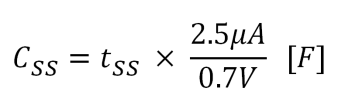where:

CSS is the capacitance required at the SS/TR pin and

tSS is the desired Soft-Start ramp time

The fastest achievable typical ramp time is 150 µs even if the external Css capacitance is lower than 680 pF or the pin is open.

#### 10.1.5 Tracking Function

If a tracking function is desired, the SS/TR pin can be used for this purpose by connecting it to an external tracking voltage. The output voltage tracks that voltage with the typical gain and offset as specified in the electrical characteristics.

When the SS/TR pin voltage is above 0.7 V, the internal voltage is clamped and the device goes to normal regulation. This works for rising and falling tracking voltages with the same behavior, as long as the input voltage is inside the recommended operating conditions. For decreasing SS/TR pin voltage in PFM mode, the device does not sink current from the output. The resulting decrease of the output voltage may therefore be slower than the SS/TR pin voltage if the load is light. When driving the SS/TR pin with an external voltage, do not exceed the voltage rating of the SS/TR pin which is VIN + 0.3 V. The SS/TR pin is internally connected with a resistor to GND when EN = 0.

If the input voltage drops below undervoltage lockout, the output voltage will go to zero, independent of the tracking voltage. Figure 4 shows how to connect devices to get ratiometric and simultaneous sequencing by using the tracking function. See also Voltage Tracking in the systems examples. SS/TR is internally clamped to approximately 3 V.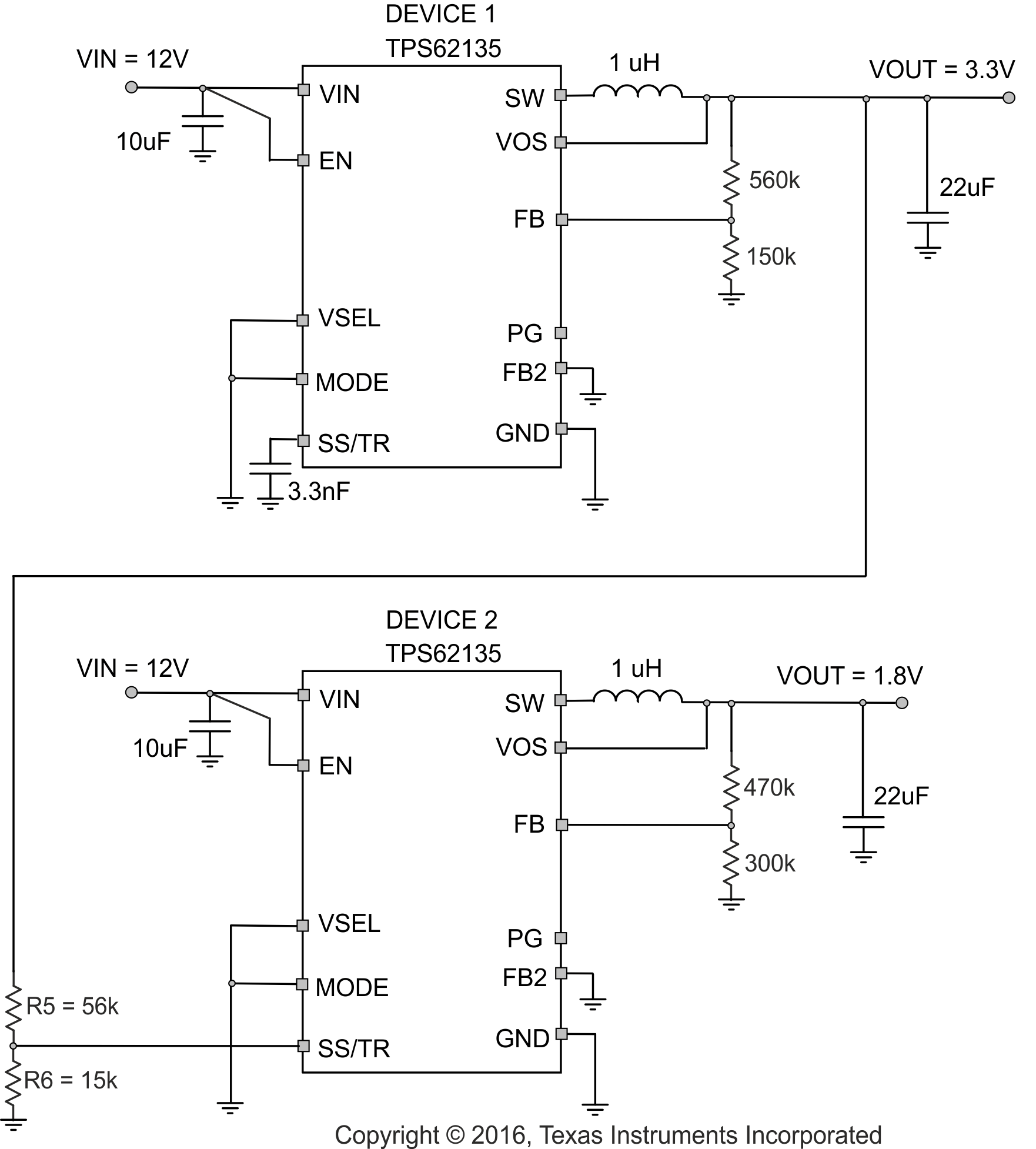Figure 4. Schematic for Ratiometric and Simultaneous Startup

The resistive divider of R5 and R6 can be used to change the ramp rate of VOUT2 to be faster, slower or the same as VOUT1.

A sequential startup is achieved by connecting the PG pin of VOUT of DEVICE 1 to the EN pin of DEVICE2. PG requires a pull-up resistor. Ratiometric start up sequence happens if both supplies are sharing the same Soft-Start capacitor. Equation 12 gives the Soft-Start time, though the SS/TR current has to be doubled. Details about these and other tracking and sequencing circuits are found in SLVA470.

Note: If the voltage at the FB pin is below its typical value of 0.7 V, the output voltage accuracy may have a wider tolerance than specified. The current of 2.5 µA out of the SS/TR pin also has an influence on the tracking function, especially for high resistive external voltage dividers on the SS/TR pin.

#### 10.1.6 Output Filter and Loop Stability

The devices of the TPS6213x family are internally compensated to be stable with L-C filter combinations corresponding to a corner frequency to be calculated with Equation 13:

Equation 13.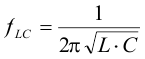Proven nominal values for inductance and ceramic capacitance are given in Table 2 and are recommended for use. Different values may work, but care has to be taken on the loop stability which is affected. More information including a detailed LC stability matrix can be found in SLVA463.

The TPS62135x devices include an internal 15 pF feedforward capacitor, connected between the VOS and FB pins. This capacitor impacts the frequency behavior and sets a pole and zero in the control loop with the resistors of the feedback divider, per equation Equation 14 and Equation 15:

Equation 14.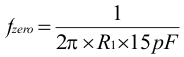Equation 15.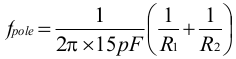Though the TPS62135x devices are stable without the pole and zero being in a particular location, adjusting their location to the specific needs of the application can provide better performance in Power Save mode and/or improved transient response. An external feedforward capacitor can also be added. A more detailed discussion on the optimization for stability versus transient response can be found in SLVA289 and SLVA466.

### 10.2 Typical Applications

#### 10.2.1 Typical Application with Adjustable Output Voltage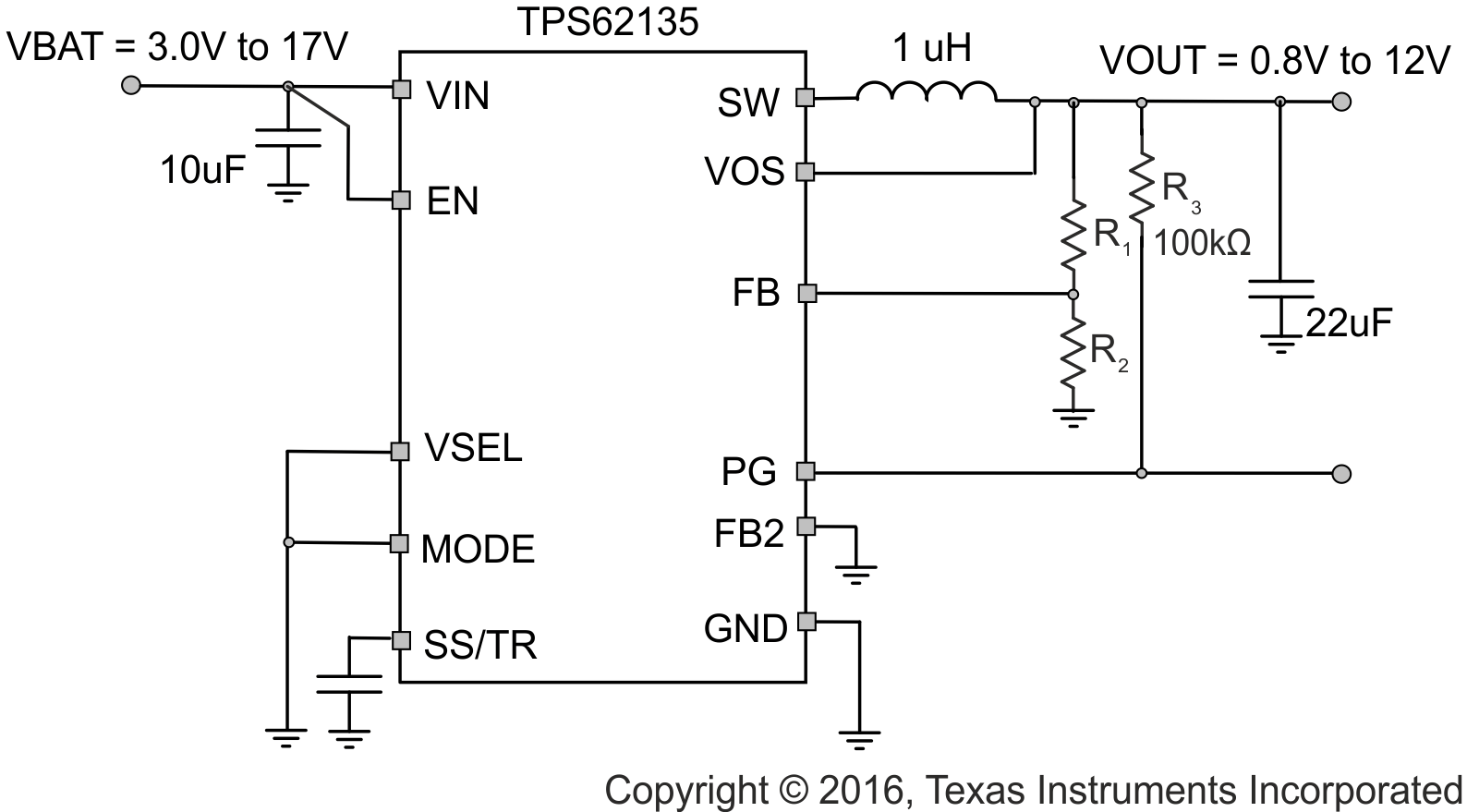Figure 5. Typical Application

#### 10.2.1.1 Design Requirements

The design guideline provides a component selection to operate the device within the recommended operating conditions. See Table 1 for the Bill of Materials used to generate the application curves.

#### 10.2.1.2 Detailed Design Procedure

Equation 16.With VFB = 0.7 V:

### Table 5. Setting the Output Voltage

NOMINAL OUTPUT VOLTAGE R1 R2 EXACT OUTPUT VOLTAGE
0.8 V 51 kΩ 360 kΩ 0.799 V
1.2 V 130 kΩ 180 kΩ 1.206 V
1.5 V 150 kΩ 130 kΩ 1.508 V
1.8 V 470 kΩ 300 kΩ 1.797 V
2.5 V 620 kΩ 240 kΩ 2.508 V
3.3 V 560 kΩ 150 kΩ 3.313 V
5 V 510 kΩ 82 kΩ 5.054 V
9 V 510 kΩ 43 kΩ 9.002 V
12 V 1000 kΩ 62 kΩ 11.99 V

#### 10.2.1.3 Application Curves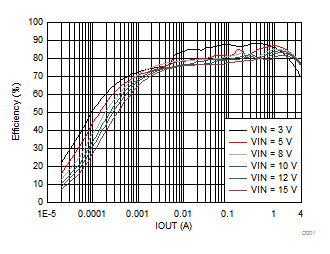Vout = 1.2 V PFM TA = 25°C
Figure 6. Efficiency vs Output Current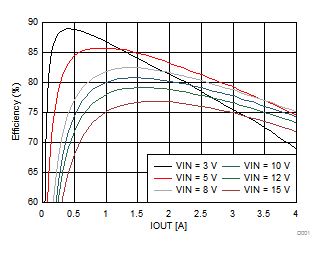Vout = 1.2 V PWM TA= 25 °C
Figure 8. Efficiency vs Output Current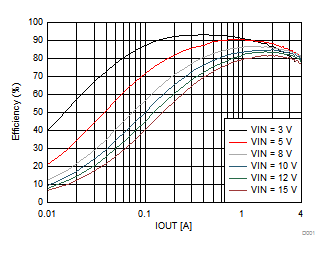Vout = 1.8 V PWM TA = 25°C
Figure 10. Efficiency vs Output Current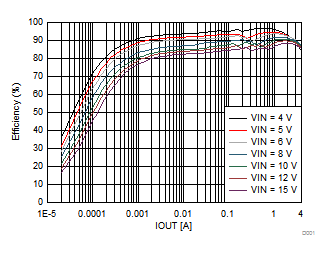Vout = 3.3 V PFM TA = 25°C
Figure 12. Efficiency vs Output Current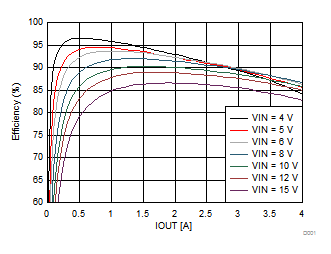Vout = 3.3 V PWM TA = 25°C
Figure 14. Efficiency vs Output Current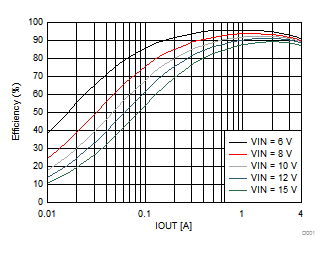Vout = 5 V PWM TA = 25°C
Figure 16. Efficiency vs Output Current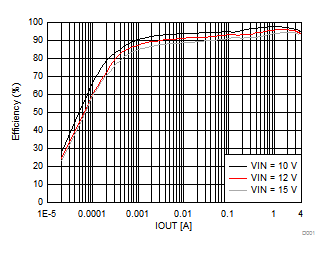Vout = 9 V PFM TA = 25°C
Figure 18. Efficiency vs Output Current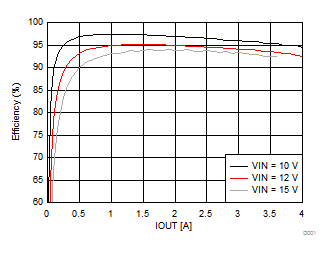Vout = 9 V PWM TA = 25°C
Figure 20. Efficiency vs Output CurrentVout = 1.2 V PWM TA = 25°C
Figure 22. Output Voltage vs Output CurrentVout = 1.8 V PWM TA = 25°C
Figure 24. Output Voltage vs Output CurrentVout = 3.3 V PWM TA = 25°C
Figure 26. Output Voltage vs Output Current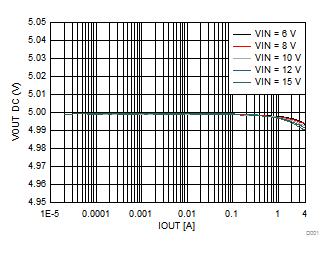Vout = 5 V PWM TA = 25°C
Figure 28. Output Voltage vs Output Current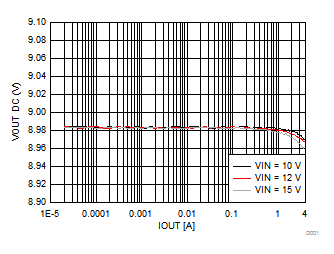Vout = 9 V PWM TA = 25°C
Figure 30. Output Voltage vs Output Current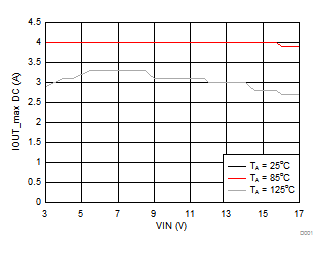Vout = 1.8 V PWM measurement taken up to 4 A
Figure 32. Maximum Output Current vs Input Voltage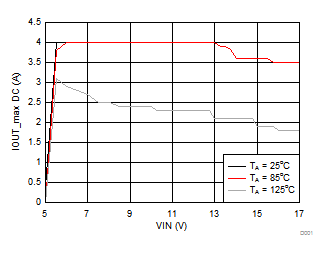Vout = 5 V PWM measurement taken up to 4 A
Figure 34. Maximum Output Current vs Input Voltage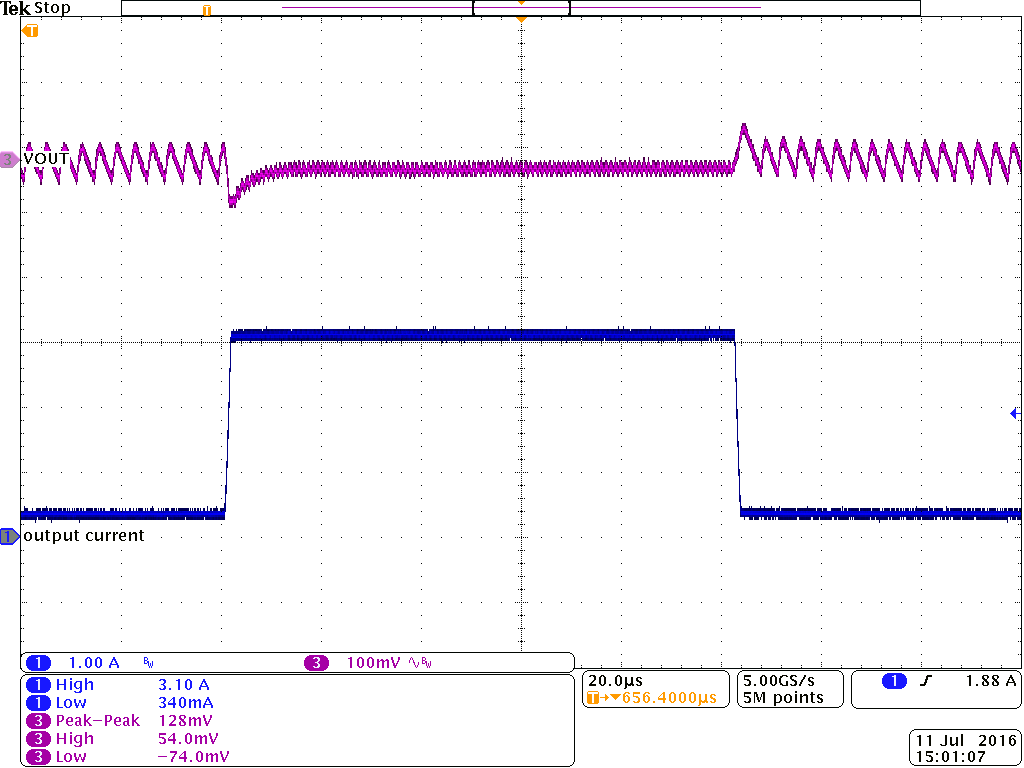Vin = 12 V; PFM Io = 350 mA to 3.1 A; Vout = 1.2 V; TA= 25 °C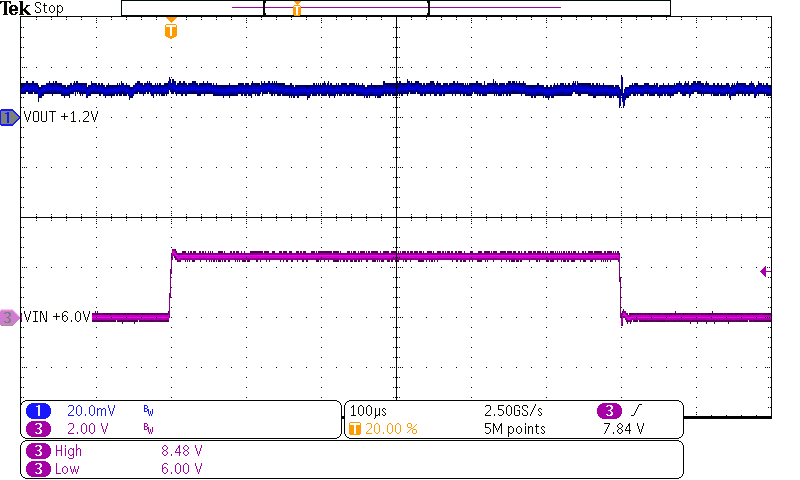Vin = 6 V to 8.4 V; PFM Io = 1 A Vout = 1.2 V TA= 25 °C
Figure 38. Line Transient Response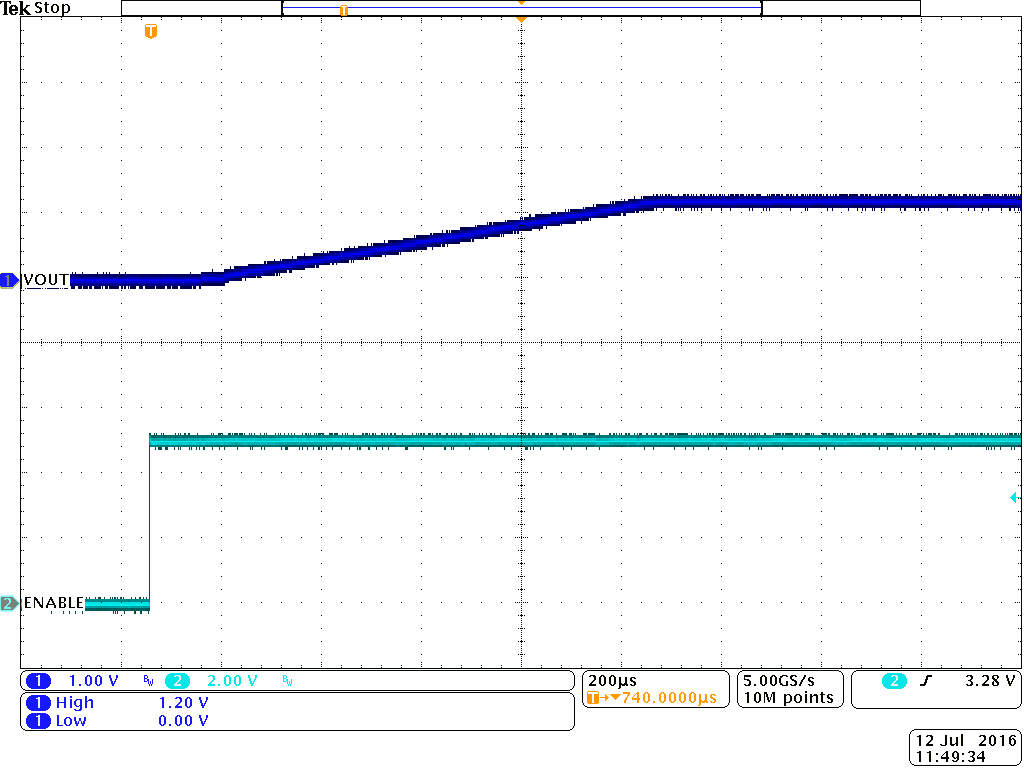Vin = 5 V; PWM Rload = 1 kΩ Vout = 1.2 V TA= 25 °C
Figure 40. Start-Up Timing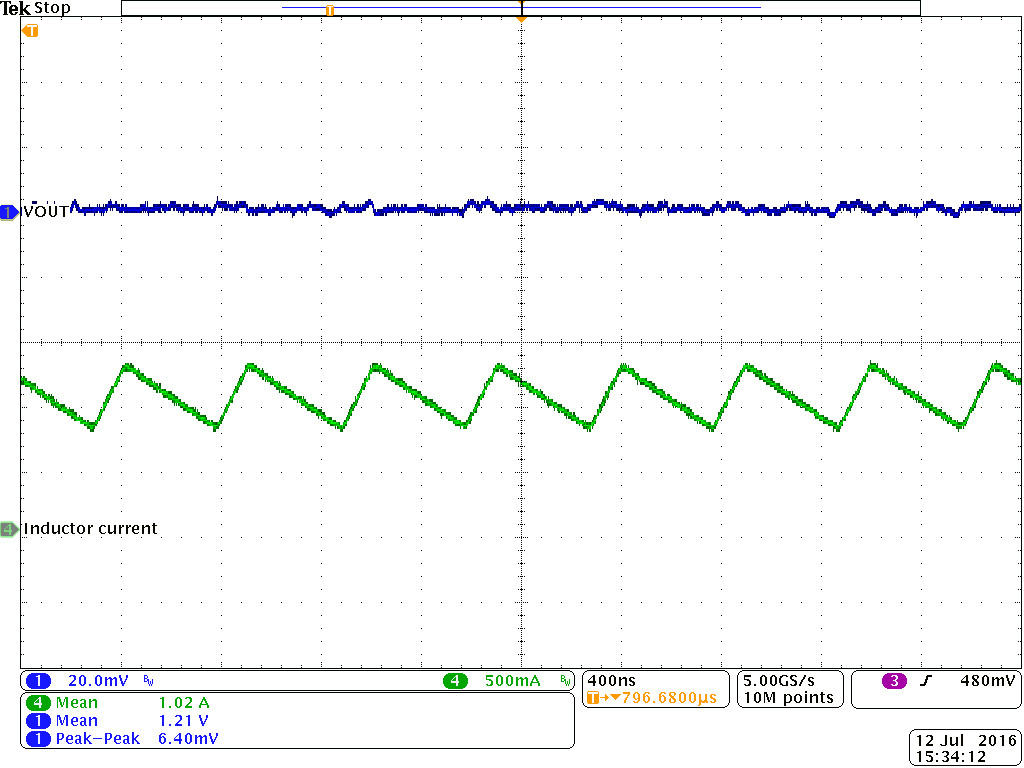Vin = 5 V; PWM Io = 1 A Vout = 1.2 V TA= 25 °C
Figure 42. Output Voltage Ripple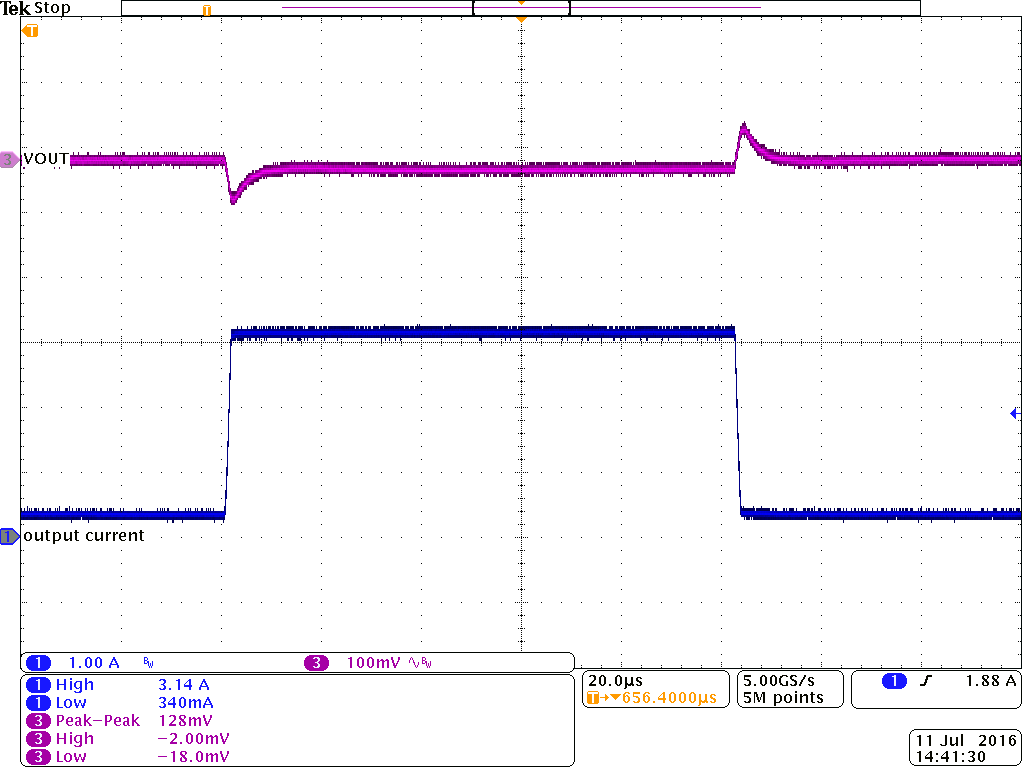Vout = 1.8 V PWM Io = 350 mA to 3.1 A TA= 25°C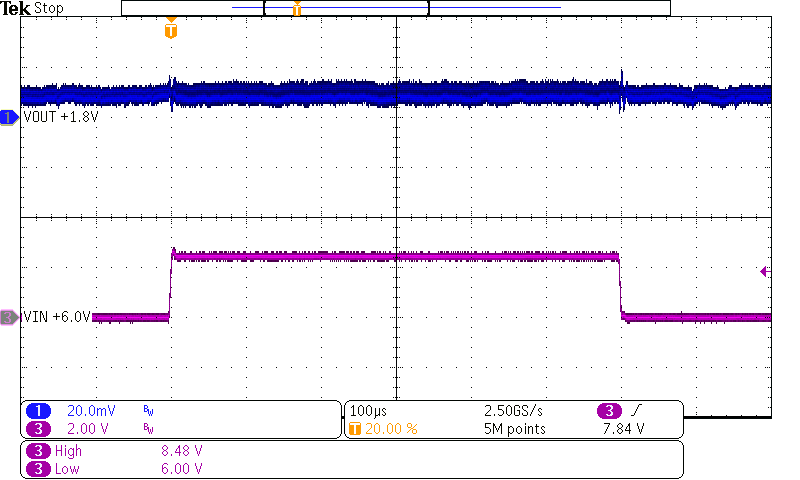Vin = 6 V to 8.4 V; PWM Io = 1 A Vout = 1.8 V TA= 25 °C
Figure 46. Line Transient ResponseVout = 1.8 V PFM Io = 0.1 A TA= 25 °C
Figure 48. Output Voltage Ripple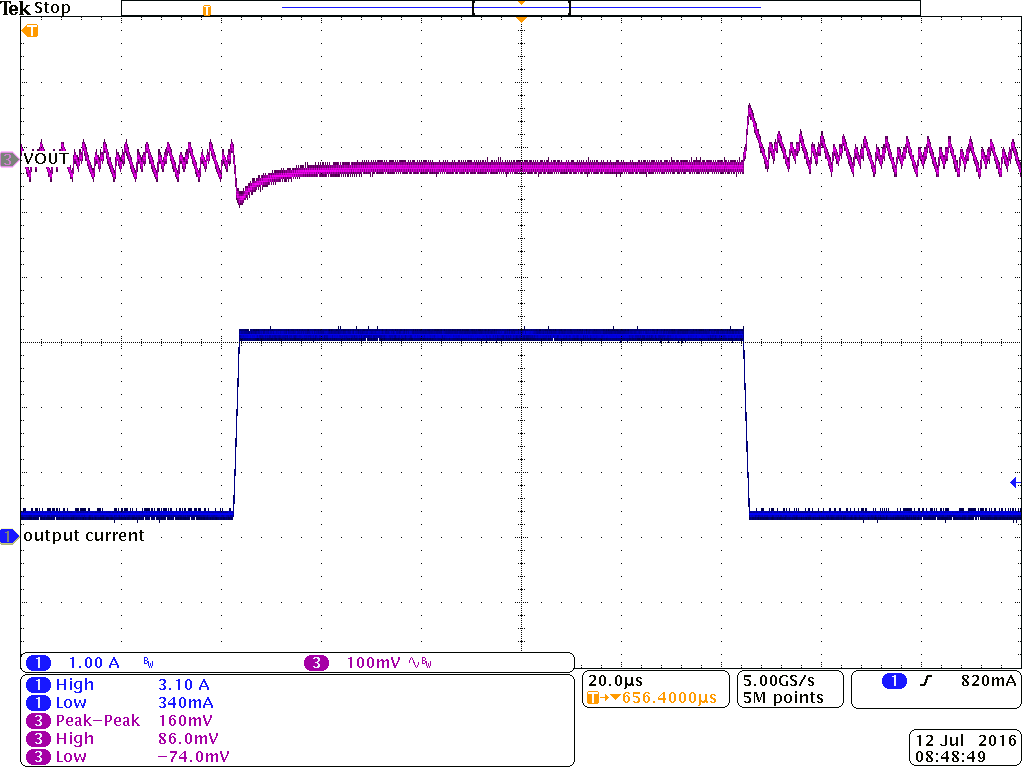Vout = 3.3 V PFM Io = 350 mA to 3.1 A TA= 25 °C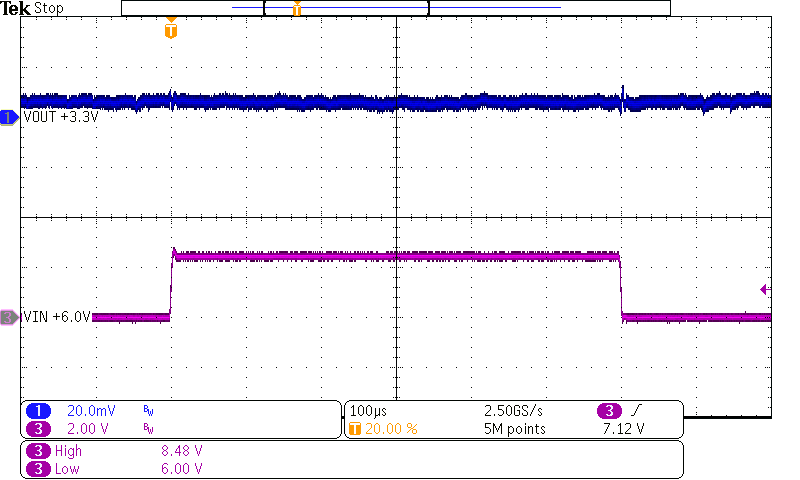Vin = 6 V to 8.4 V; PFM Io = 1 A Vout = 3.3 V TA= 25 °C
Figure 52. Line Transient Response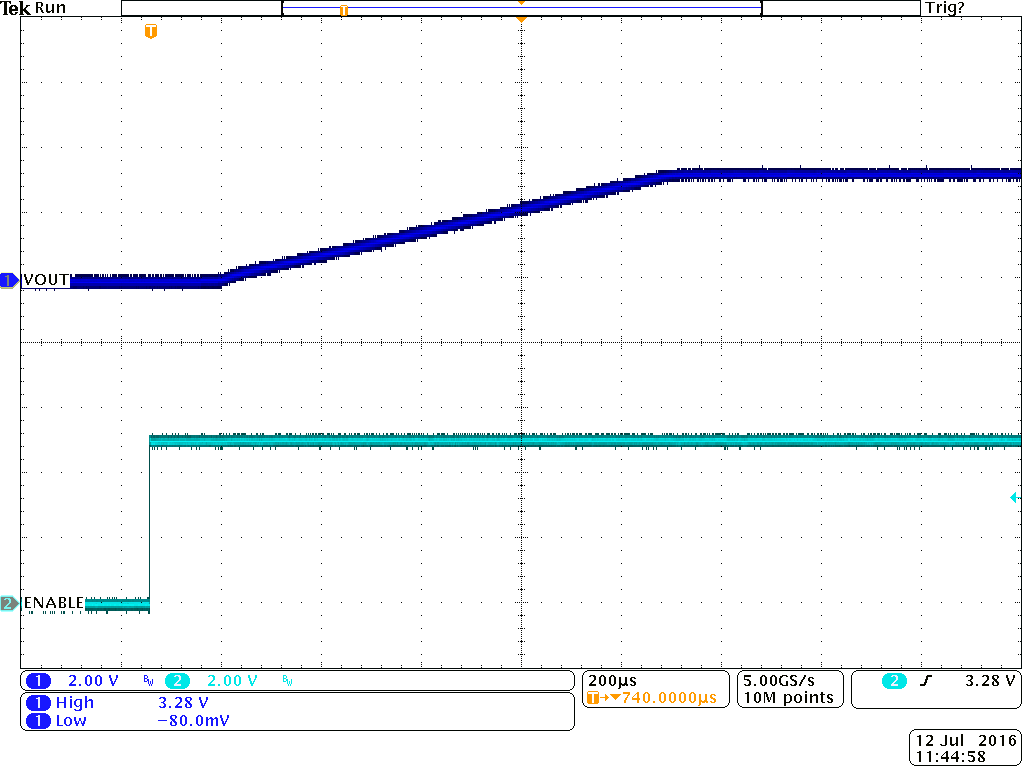Vout = 3.3 V PWM Rload = 1 kΩ TA= 25 °C
Figure 54. Start-Up Timing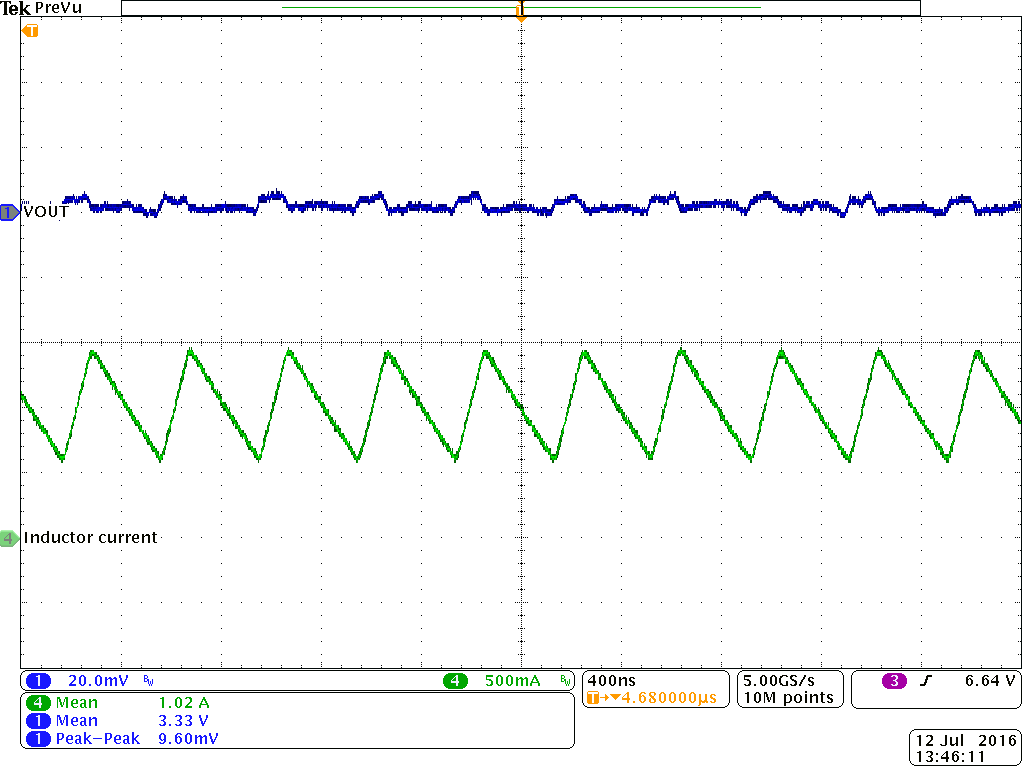Vout = 3.3 V PWM Io = 1 A TA= 25 °C
Figure 56. Output Voltage Ripple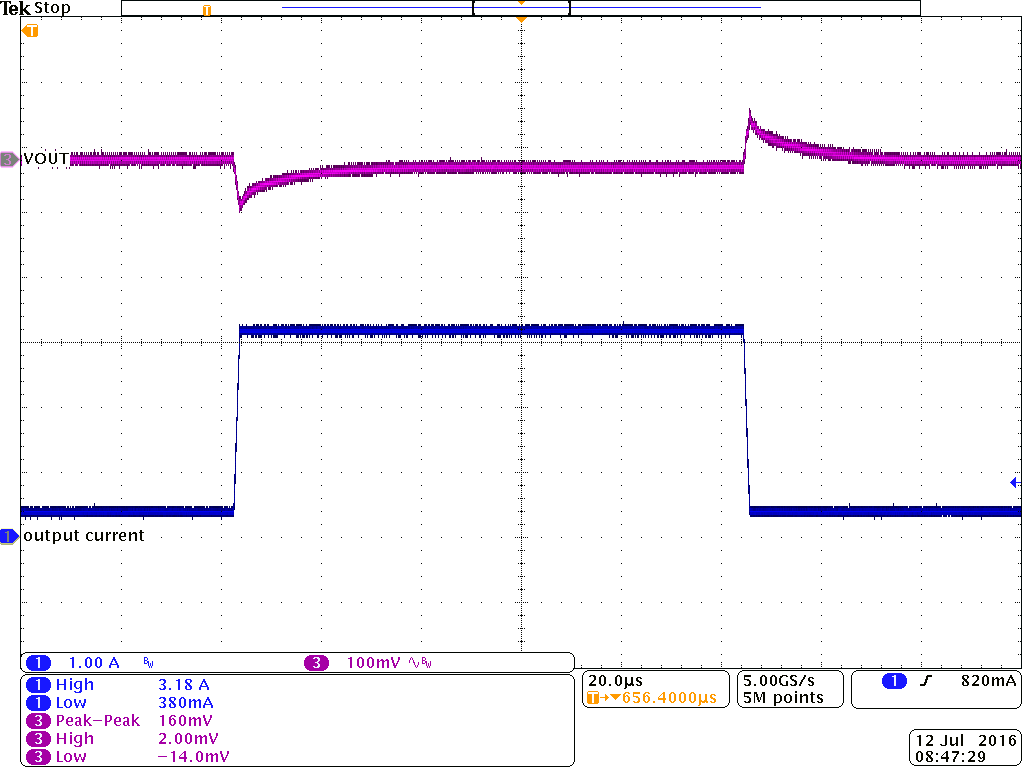Vout = 5 V PWM Io = 350 mA to 3.1 A TA= 25 °C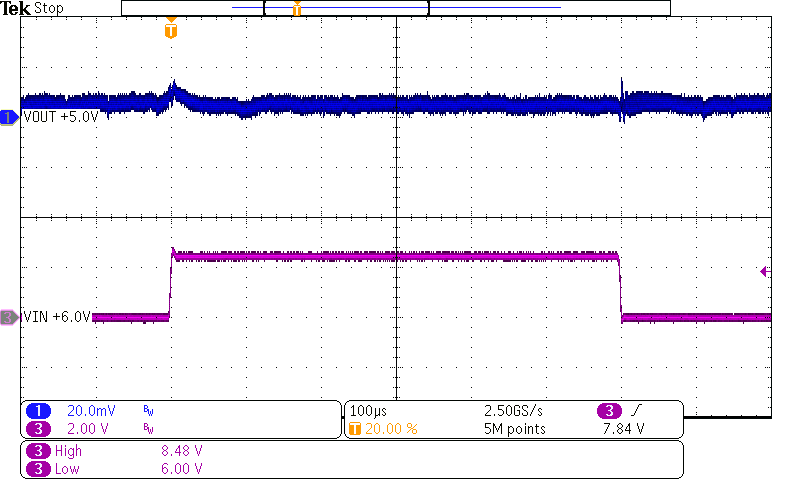Vin = 6 V to 8.4 V; PWM Io = 1 A Vout = 5 V TA= 25 °C
Figure 60. Line Transient ResponseVout = 5 V PFM Io = 0.1 A TA= 25 °C
Figure 62. Output Voltage Ripple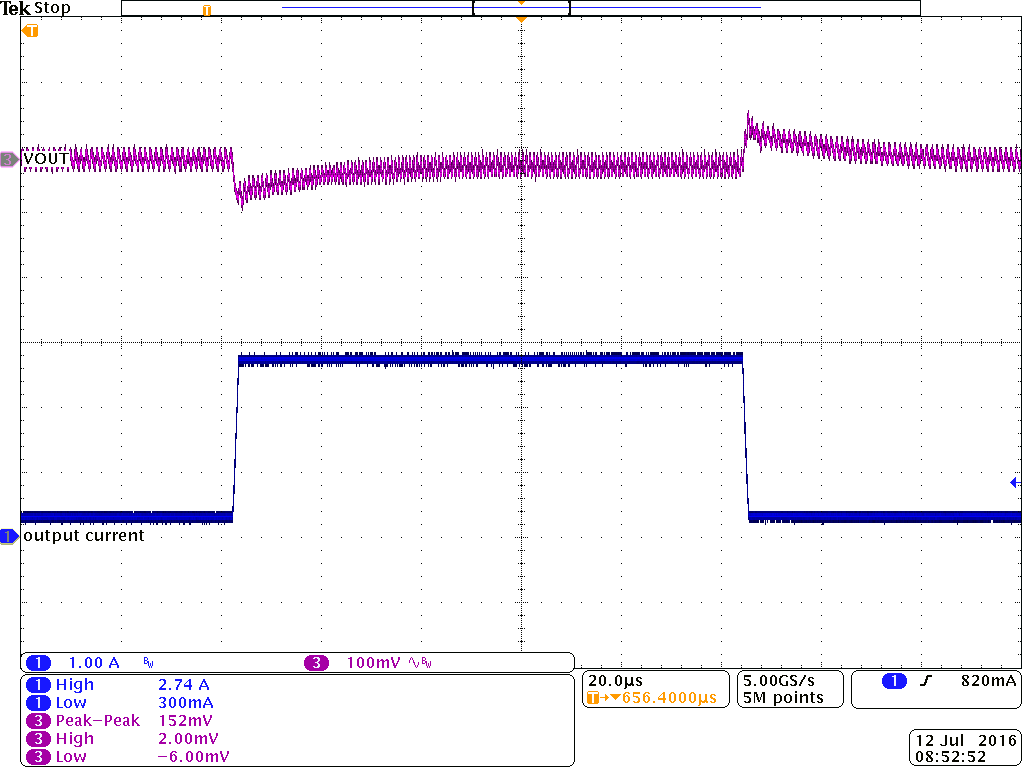Vout = 9 V PFM Io = 300 mA to 2.7 A TA= 25 °C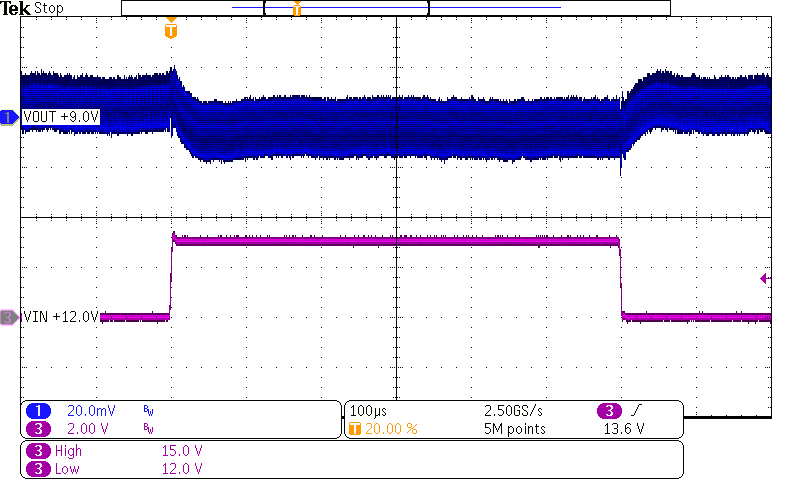Vin = 12 V to 15 V; PFM Io = 1 A Vout = 9 V TA= 25 °C
Figure 66. Line Transient Response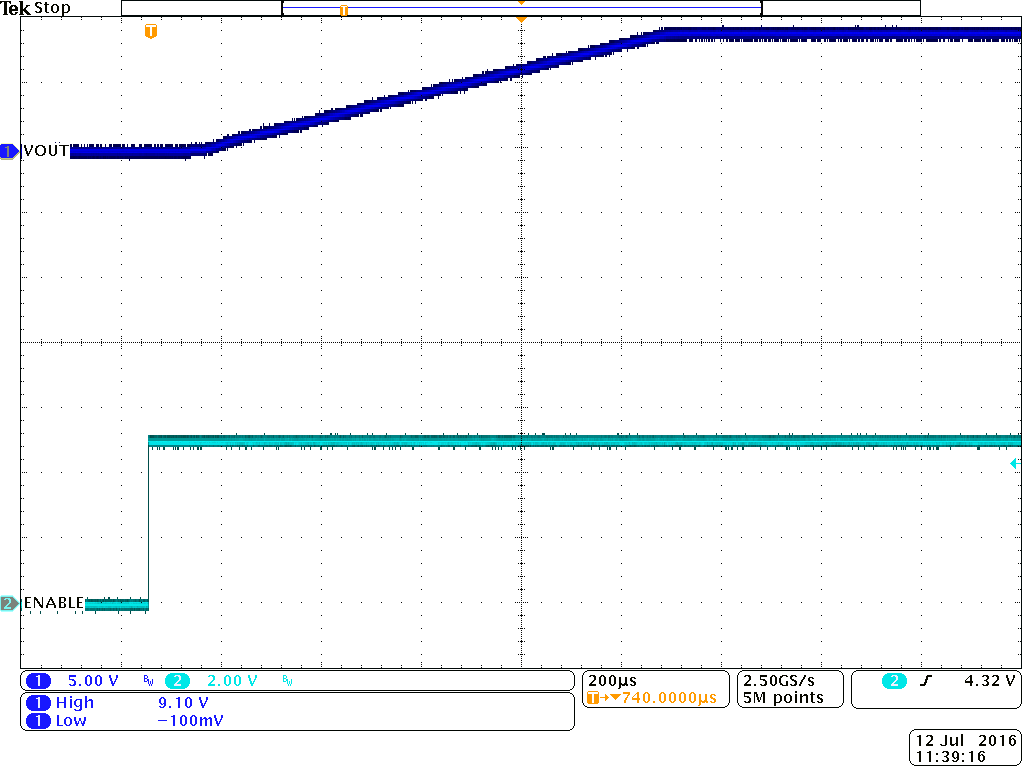Vout = 9 V PWM Rload = 1 kΩ TA= 25 °C
Figure 68. Start-Up Timing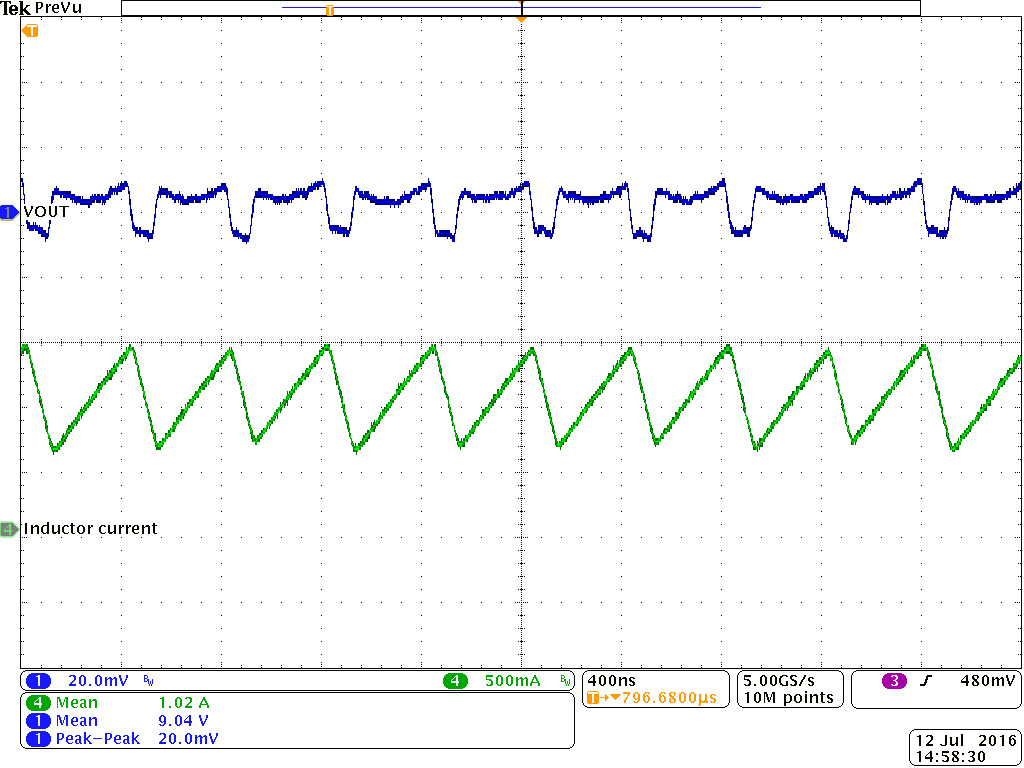Vout = 9 V PWM Io = 1 A TA= 25 °C
Figure 70. Output Voltage Ripple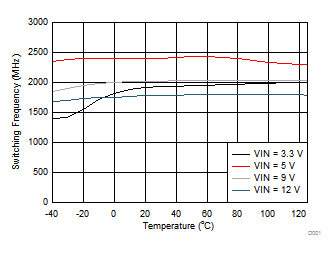Vout = 1.2 V PWM Iout = 1 A
Figure 72. Switching Frequency vs Junction Temperature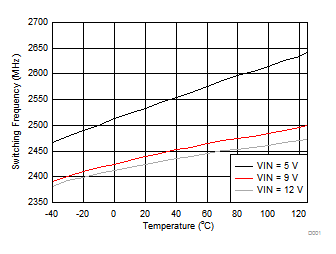Vout = 1.8 V PWM Iout = 1 A
Figure 74. Switching Frequency vs Junction Temperature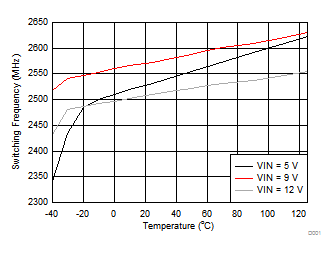Vout = 3.3 V PWM Iout = 1 A
Figure 76. Switching Frequency vs Junction Temperature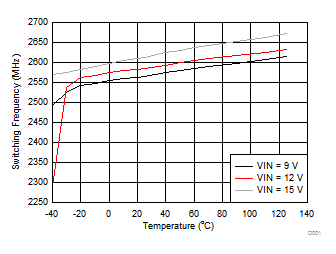Vout = 5.0 V PWM Iout = 1 A
Figure 78. Switching Frequency vs Junction Temperature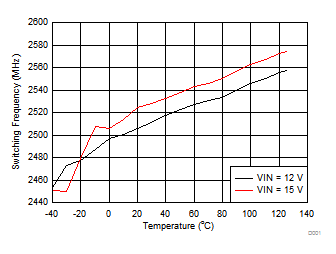Vout = 9.0 V PWM Iout = 1 A
Figure 80. Switching Frequency vs Junction Temperature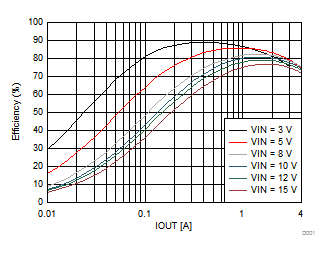Vout = 1.2 V PWM TA = 25°C
Figure 7. Efficiency vs Output Current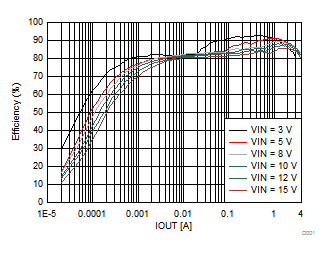Vout = 1.8 V PFM TA = 25°C
Figure 9. Efficiency vs Output Current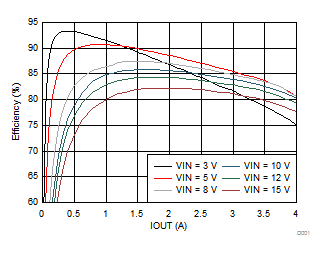Vout = 1.8 V PWM TA= 25 °C
Figure 11. Efficiency vs Output Current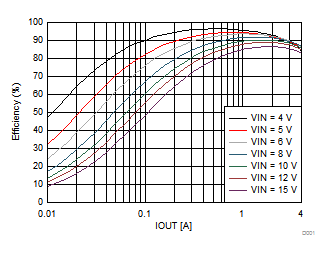Vout = 3.3 V PWM TA = 25°C
Figure 13. Efficiency vs Output Current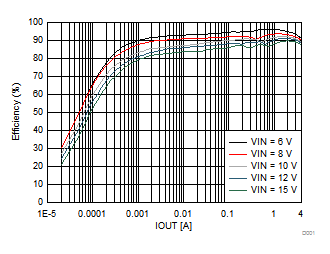Vout = 5 V PFM TA = 25°C
Figure 15. Efficiency vs Output Current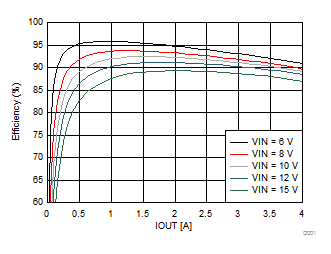Vout = 5 V PWM TA = 25°C
Figure 17. Efficiency vs Output Current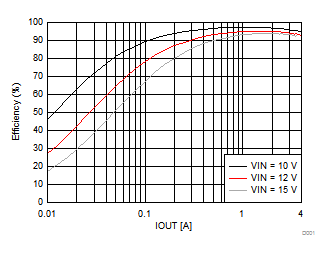Vout = 9 V PWM TA = 25°C
Figure 19. Efficiency vs Output Current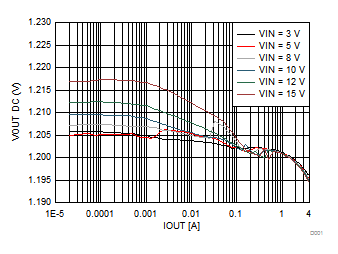Vout = 1.2 V PFM TA = 25°C
Figure 21. Output Voltage vs Output Current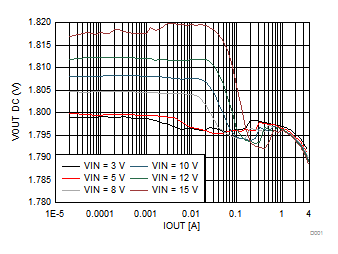Vout = 1.8 V PFM TA = 25°C
Figure 23. Output Voltage vs Output Current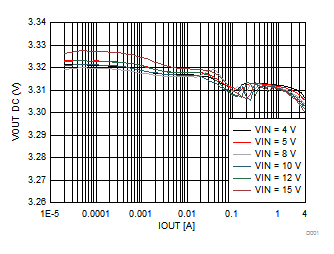Vout = 3.3 V PFM TA = 25°C
Figure 25. Output Voltage vs Output Current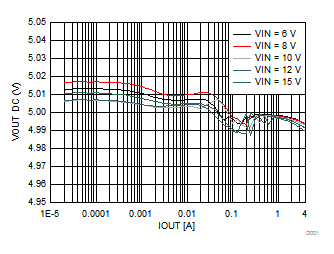Vout = 5 V PFM TA = 25°C
Figure 27. Output Voltage vs Output Current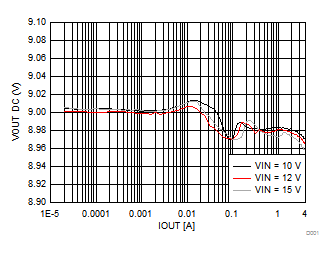Vout = 9 V PFM TA = 25°C
Figure 29. Output Voltage vs Output Current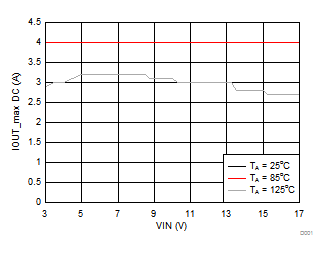Vout = 1.2 V PWM measurement taken up to 4 A Curves for TA= 25 °C and TA= 85 °C overlap
Figure 31. Maximum Output Current vs Input Voltage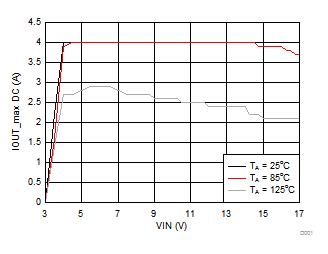Vout = 3.3 V PWM measurement taken up to 4 A
Figure 33. Maximum Output Current vs Input Voltage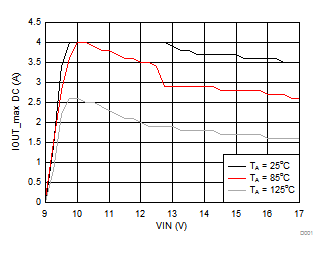Vout = 9 V PWM measurement taken up to 4 A
Figure 35. Maximum Output Current vs Input Voltage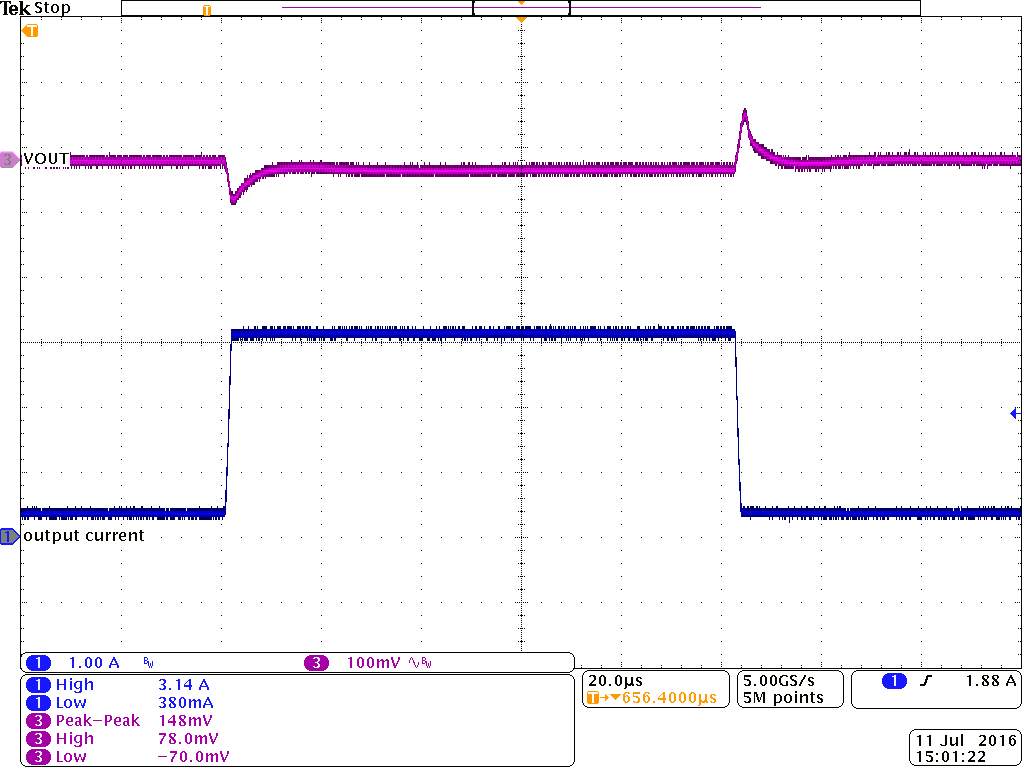Vin = 12 V; PWM Io = 350 mA to 3.1 A Vout = 1.2 V TA= 25 °C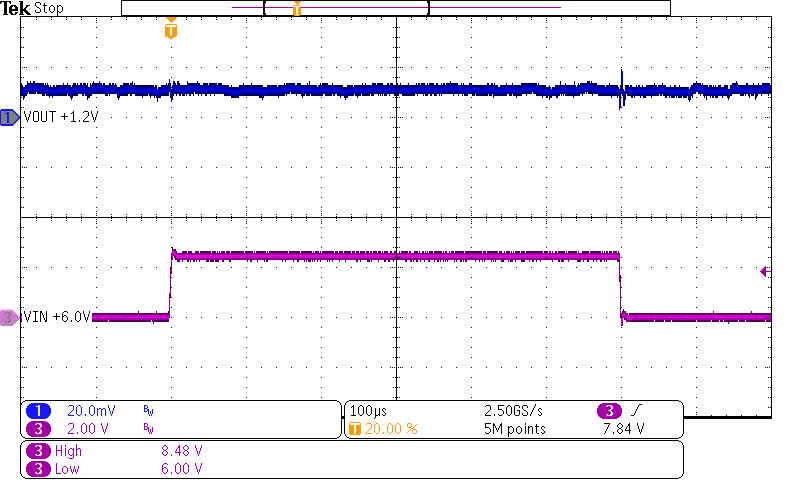Vin = 6 V to 8.4 V; PWM Io = 1 A Vout = 1.2 V TA= 25 °C
Figure 39. Line Transient Response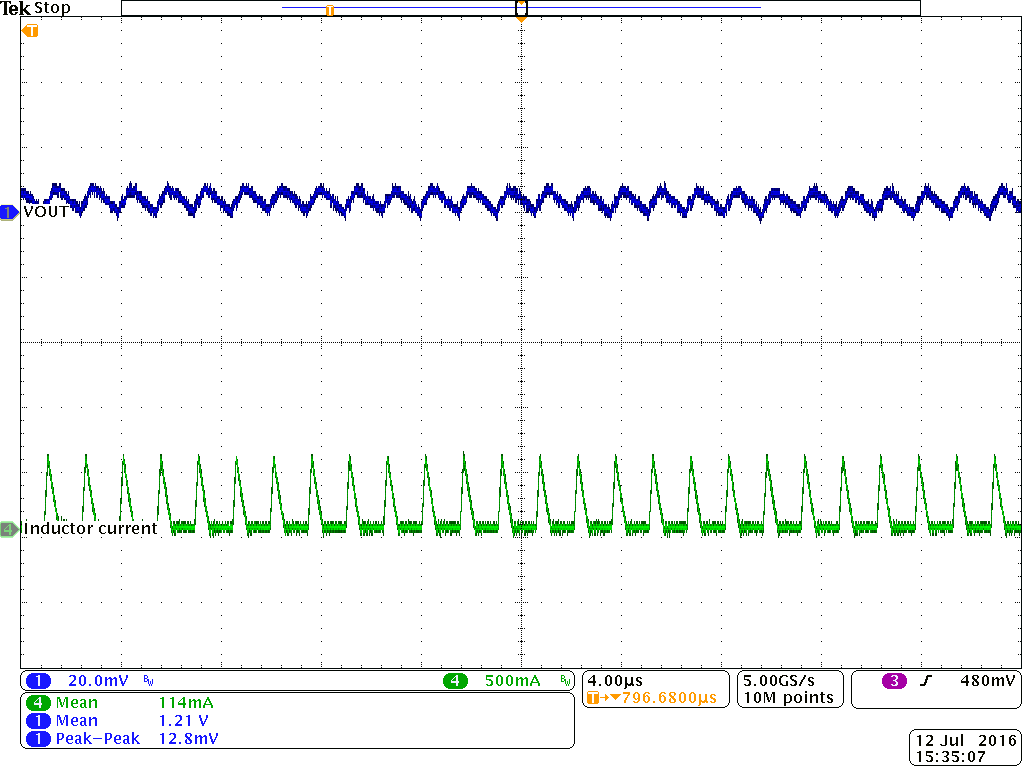Vin = 5 V; PFM Io = 0.1 A Vout = 1.2 V TA= 25 °C
Figure 41. Output Voltage Ripple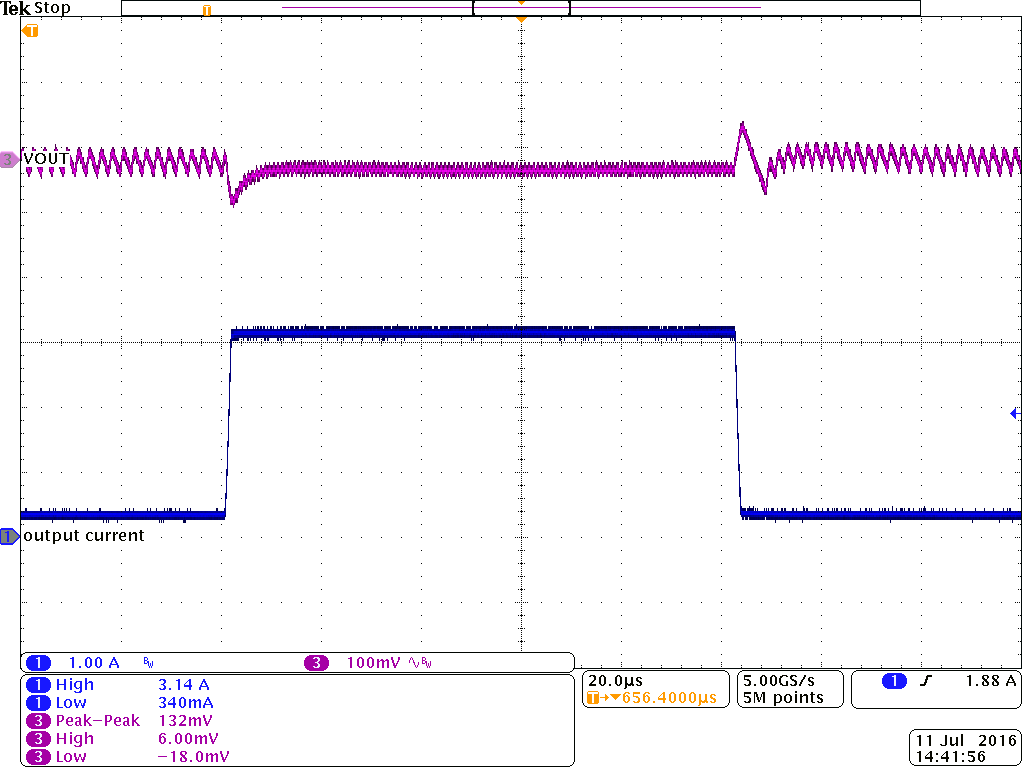Vout = 1.8 V PFM Io = 350 mA to 3.1 A TA= 25 °C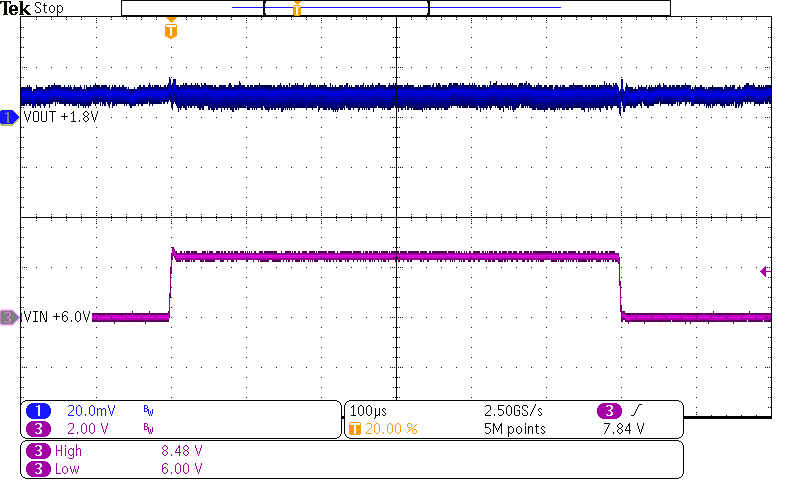Vin = 6 V to 8.4 V; PFM Io = 1 A Vout = 1.8 V TA= 25 °C
Figure 45. Line Transient Response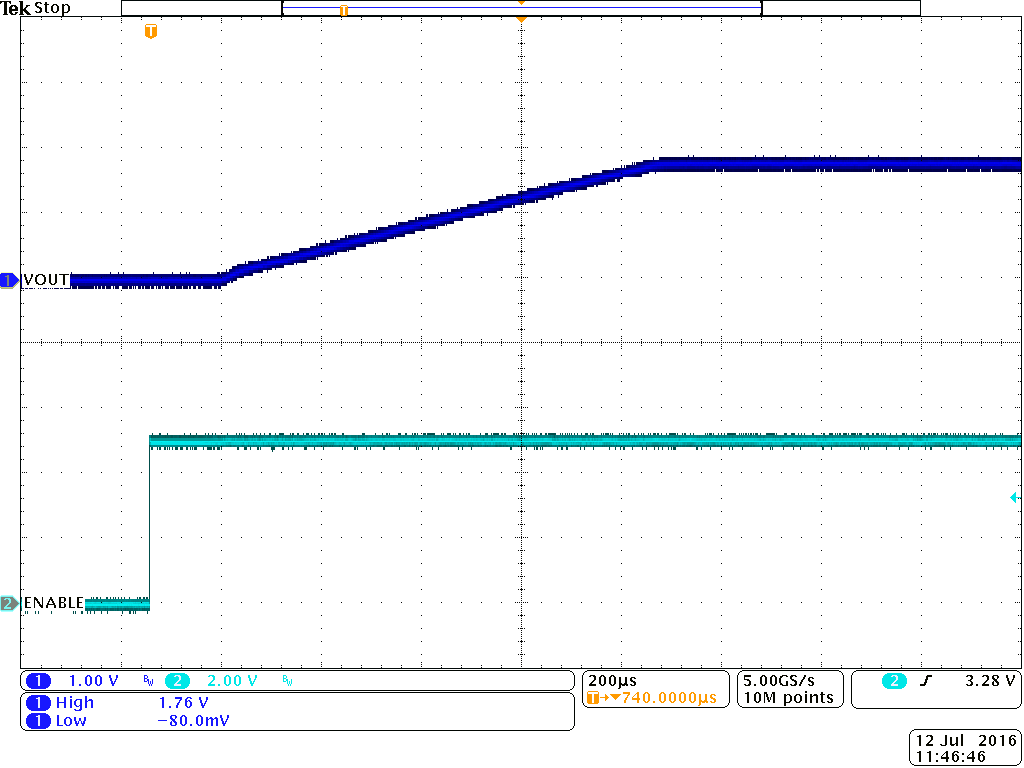Vout = 1.8 V PWM Rload = 1 kΩ TA= 25 °C
Figure 47. Start-Up Timing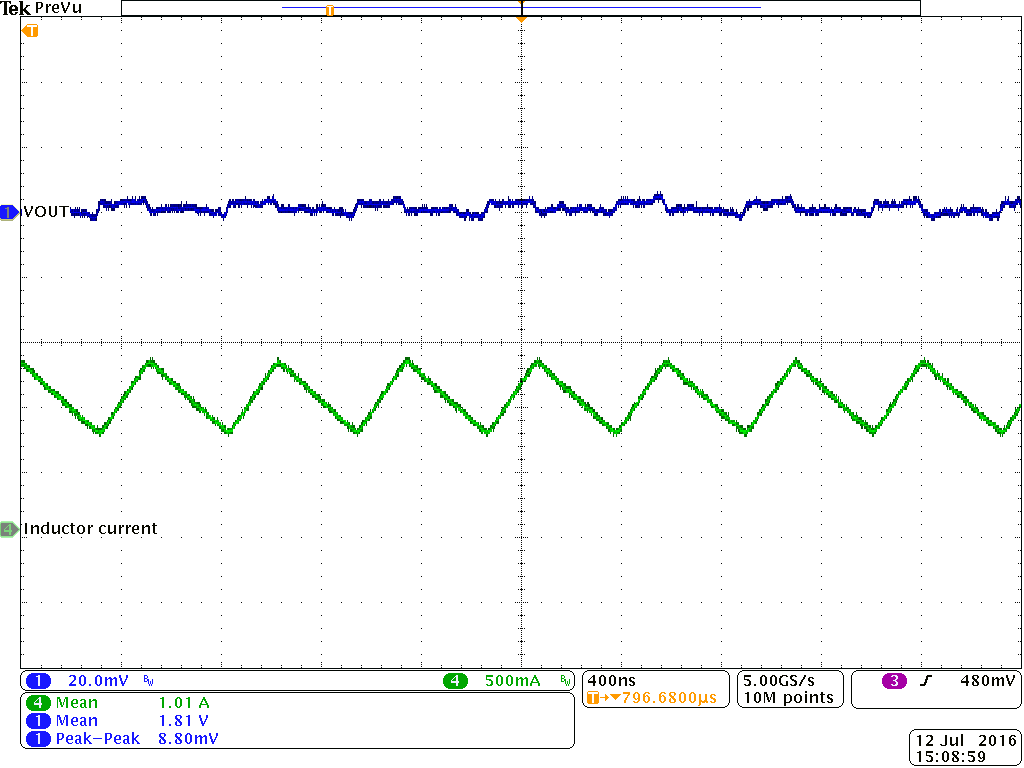Vout = 1.8 V PWM Io = 1 A TA= 25 °C
Figure 49. Output Voltage Ripple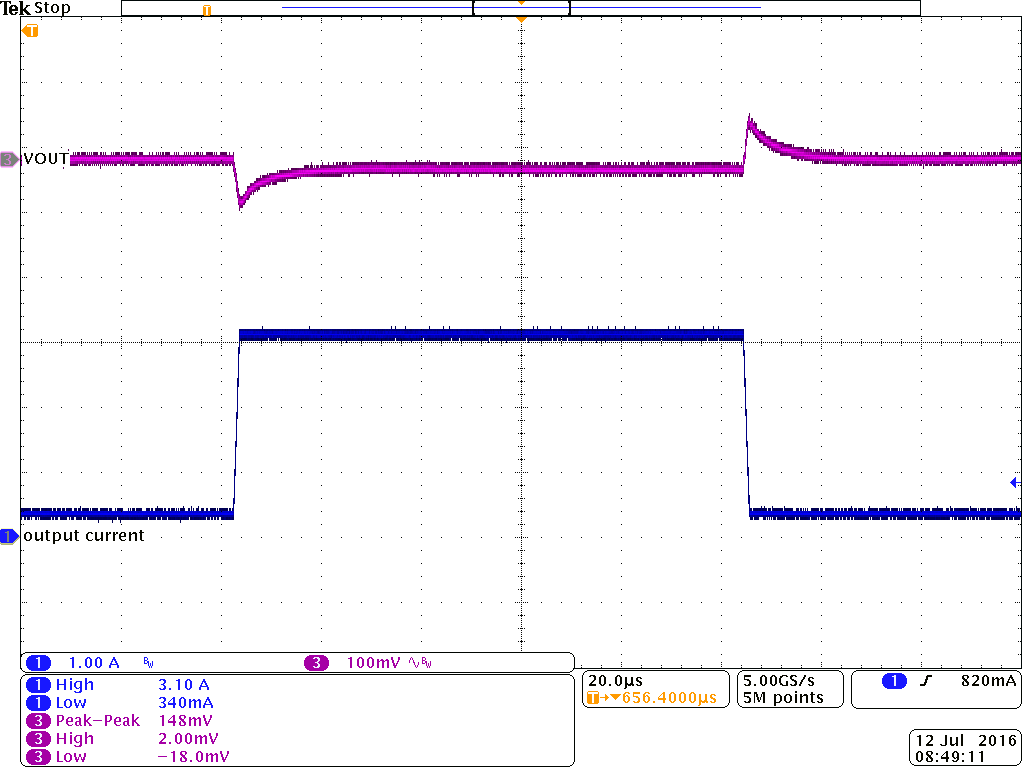Vout = 3.3 V PWM Io = 350 mA to 3.1 A TA= 25 °C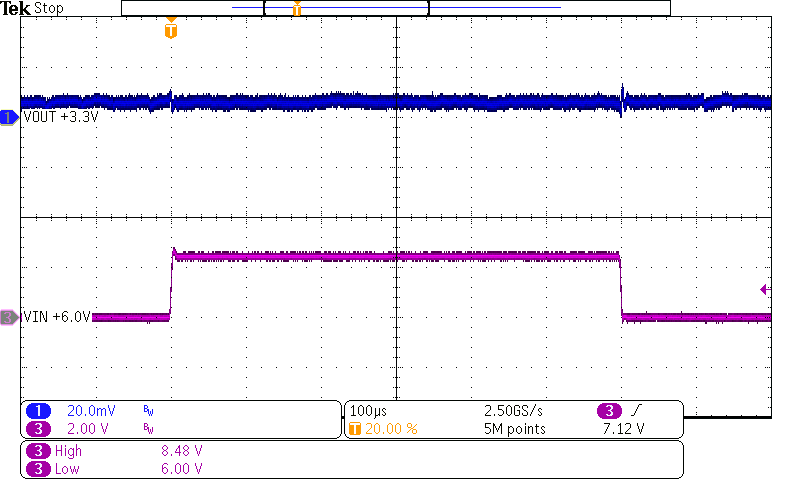Vin = 6 V to 8.4 V; PWM Io = 1 A Vout = 3.3 V TA= 25 °C
Figure 53. Line Transient Response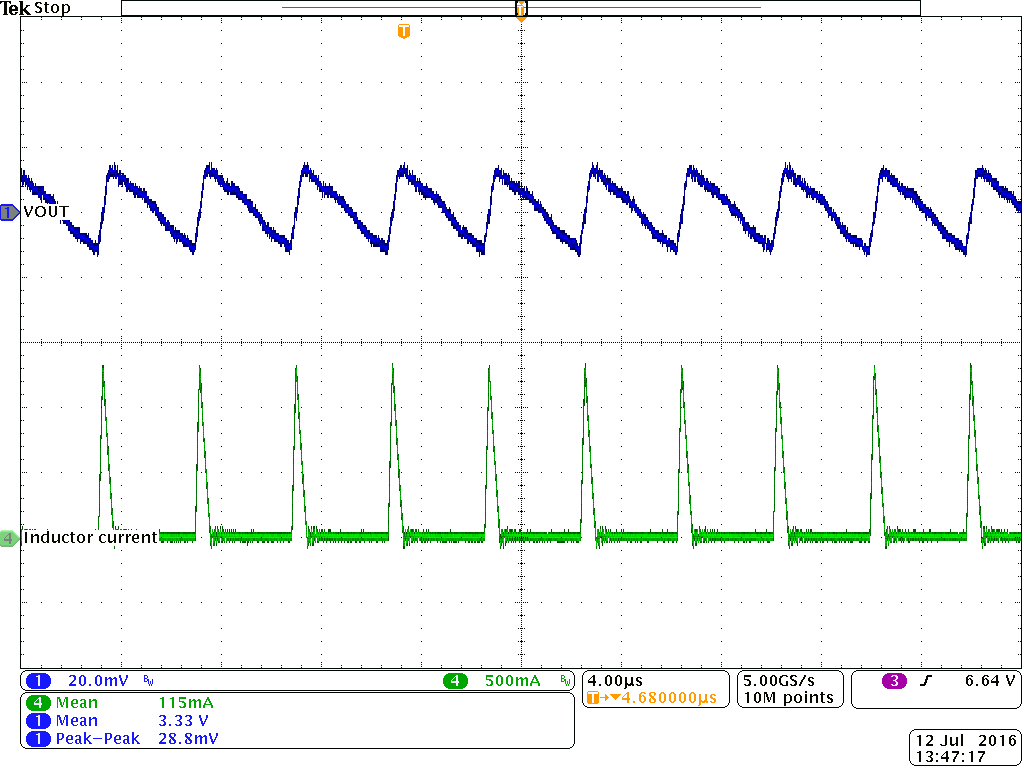Vout = 3.3 V PFM Io = 0.1 A TA= 25 °C
Figure 55. Output Voltage Ripple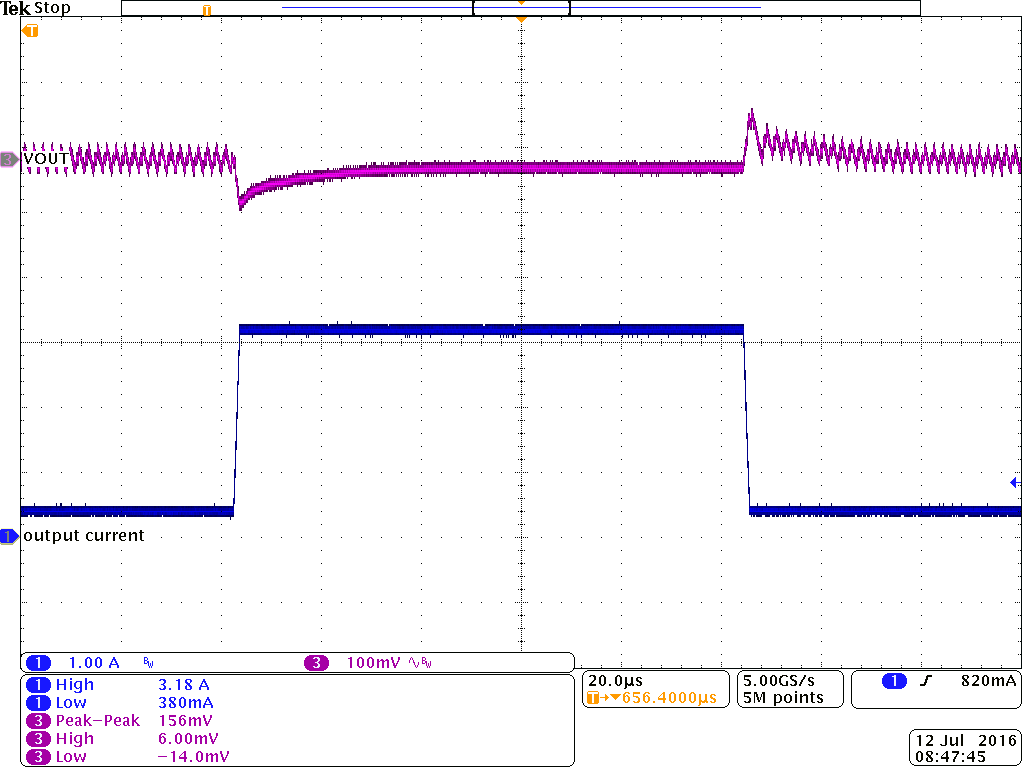Vout = 5 V PFM Io = 350 mA to 3.1 A TA= 25 °C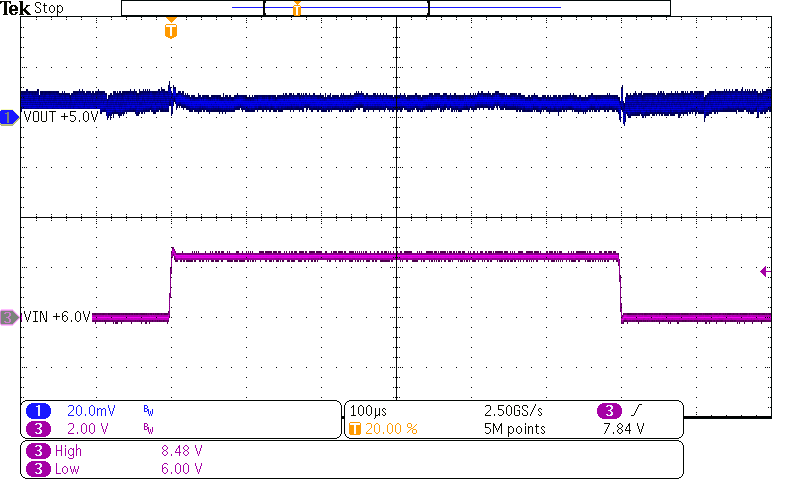Vin = 6 V to 8.4 V; PFM Io = 1 A Vout = 5 V TA= 25 °C
Figure 59. Line Transient Response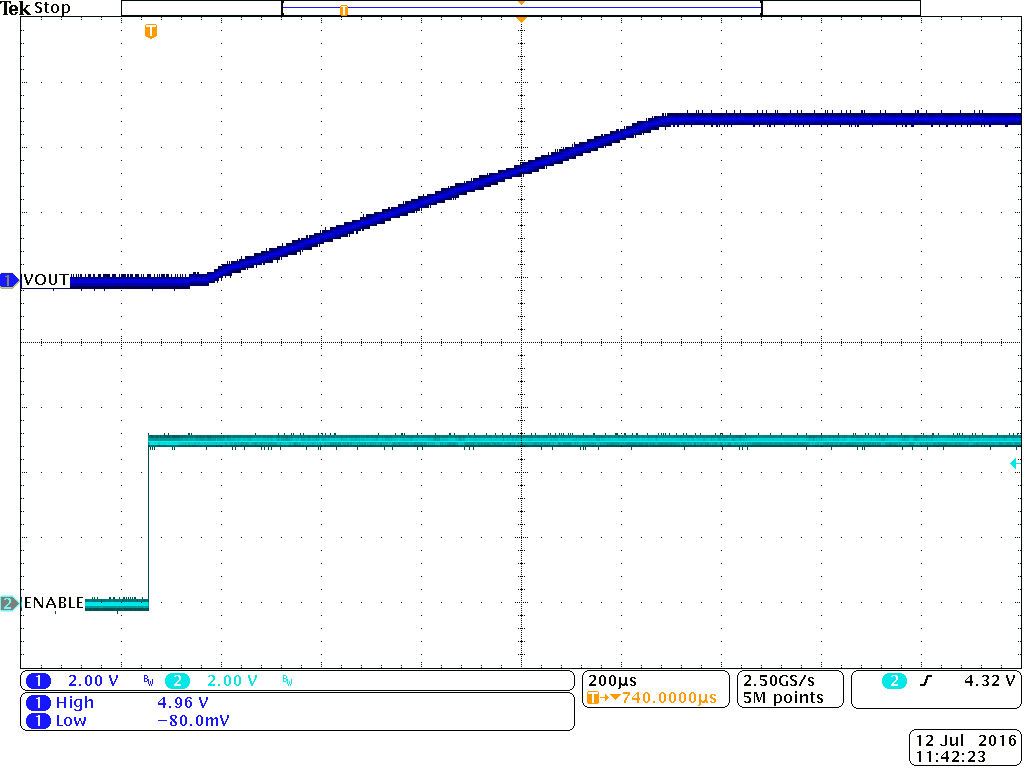Vout = 5 V PWM Rload = 1 kΩ TA= 25 °C
Figure 61. Start-Up Timing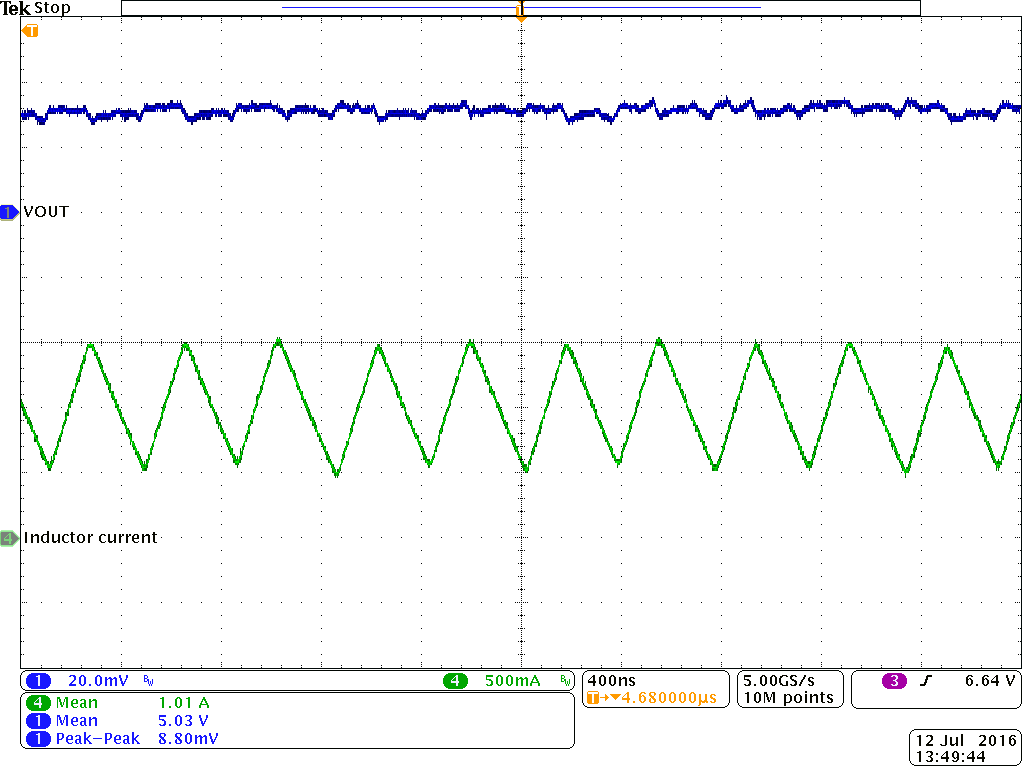Vout = 5 V PWM Io = 1 A TA= 25 °C
Figure 63. Output Voltage Ripple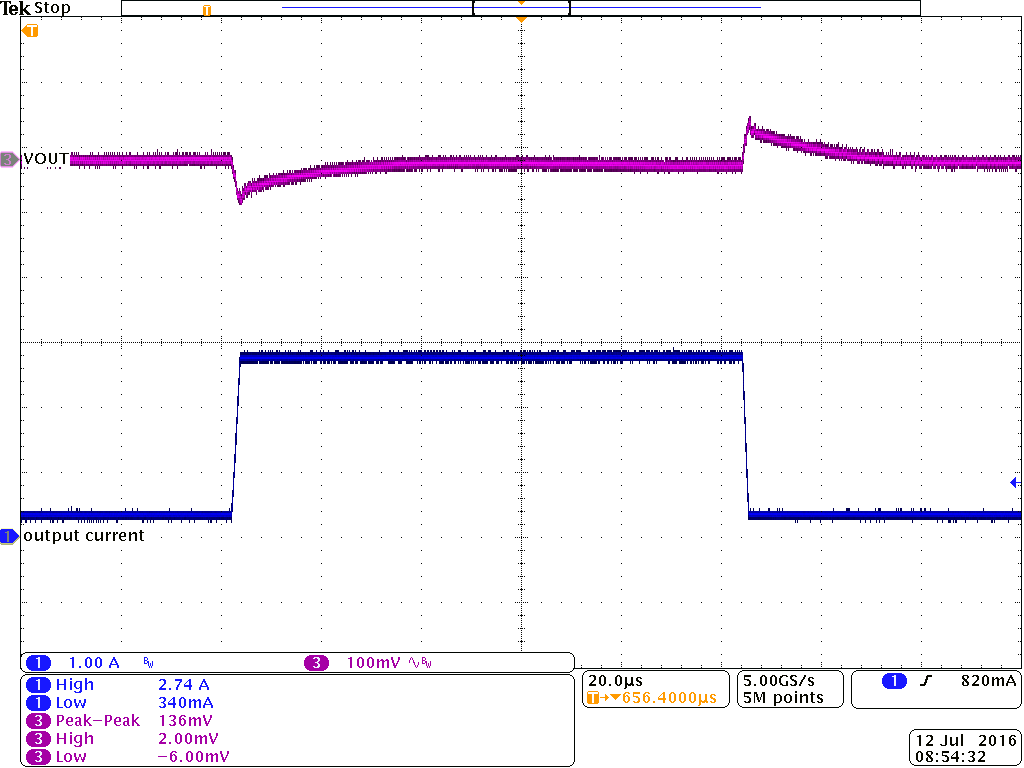Vout = 9 V PWM Io = 300 mA to 2.7 A TA= 25 °C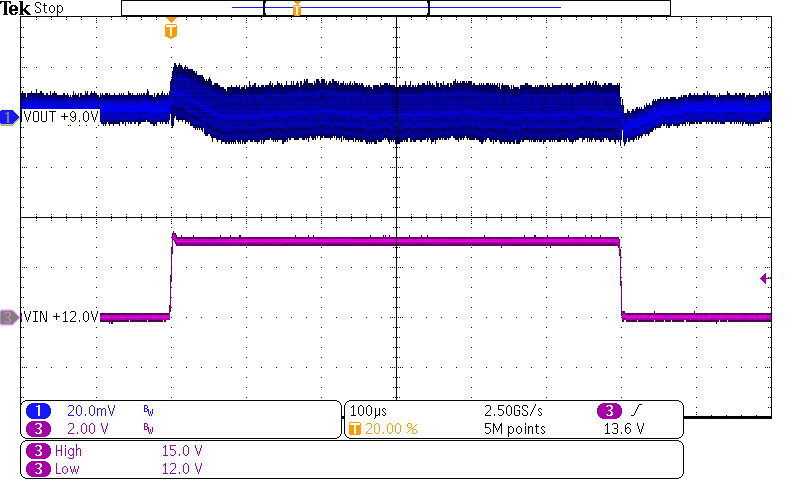Vin = 12 V to 15 V; PWM Io = 1 A Vout = 9 V TA= 25 °C
Figure 67. Line Transient Response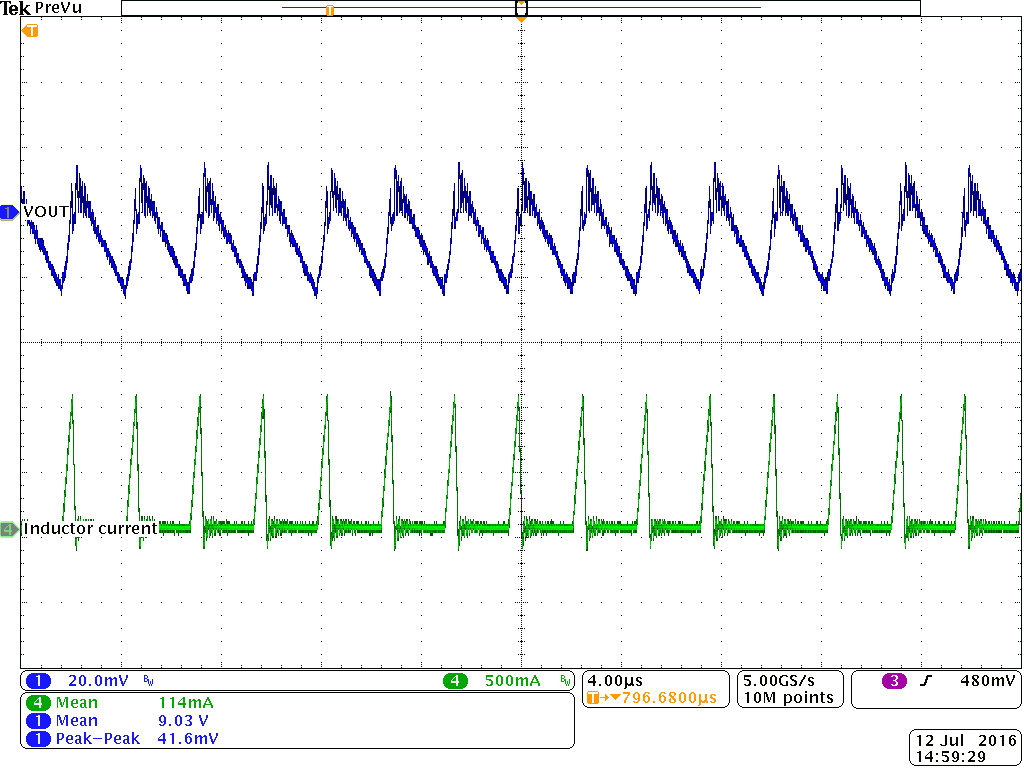Vout = 9 V PFM Io = 0.1 A TA= 25 °C
Figure 69. Output Voltage Ripple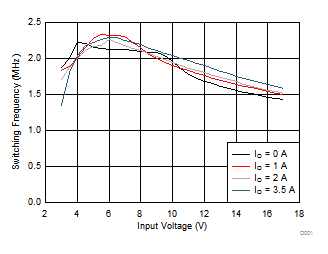Vout = 1.2 V PWM TA= 25 °C
Figure 71. Switching Frequency vs Input Voltage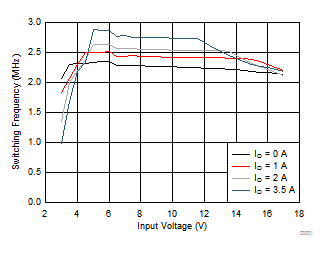Vout = 1.8 V PWM TA= 25 °C
Figure 73. Switching Frequency vs Input Voltage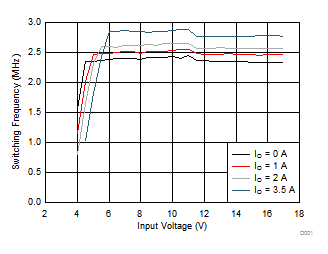Vout = 3.3 V PWM TA= 25 °C
Figure 75. Switching Frequency vs Input Voltage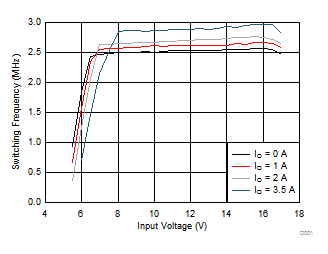Vout = 5 V PWM TA= 25 °C
Figure 77. Switching Frequency vs Input VoltageVout = 9 V PWM TA= 25 °C
Figure 79. Switching Frequency vs Input Voltage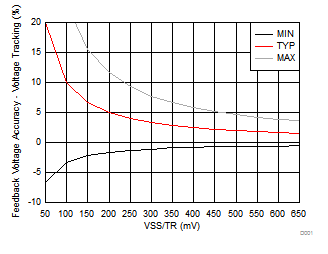Figure 81. Feedback Voltage Accuracy with Voltage Tracking vs Voltage at SS/TR Pin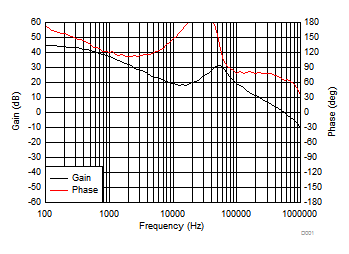Vout = 3.3 V Vin = 12 V TA= 25 °C Io = 3.5 A
Figure 82. Bode Plot

#### 10.2.2 Typical Application using VSEL and FB2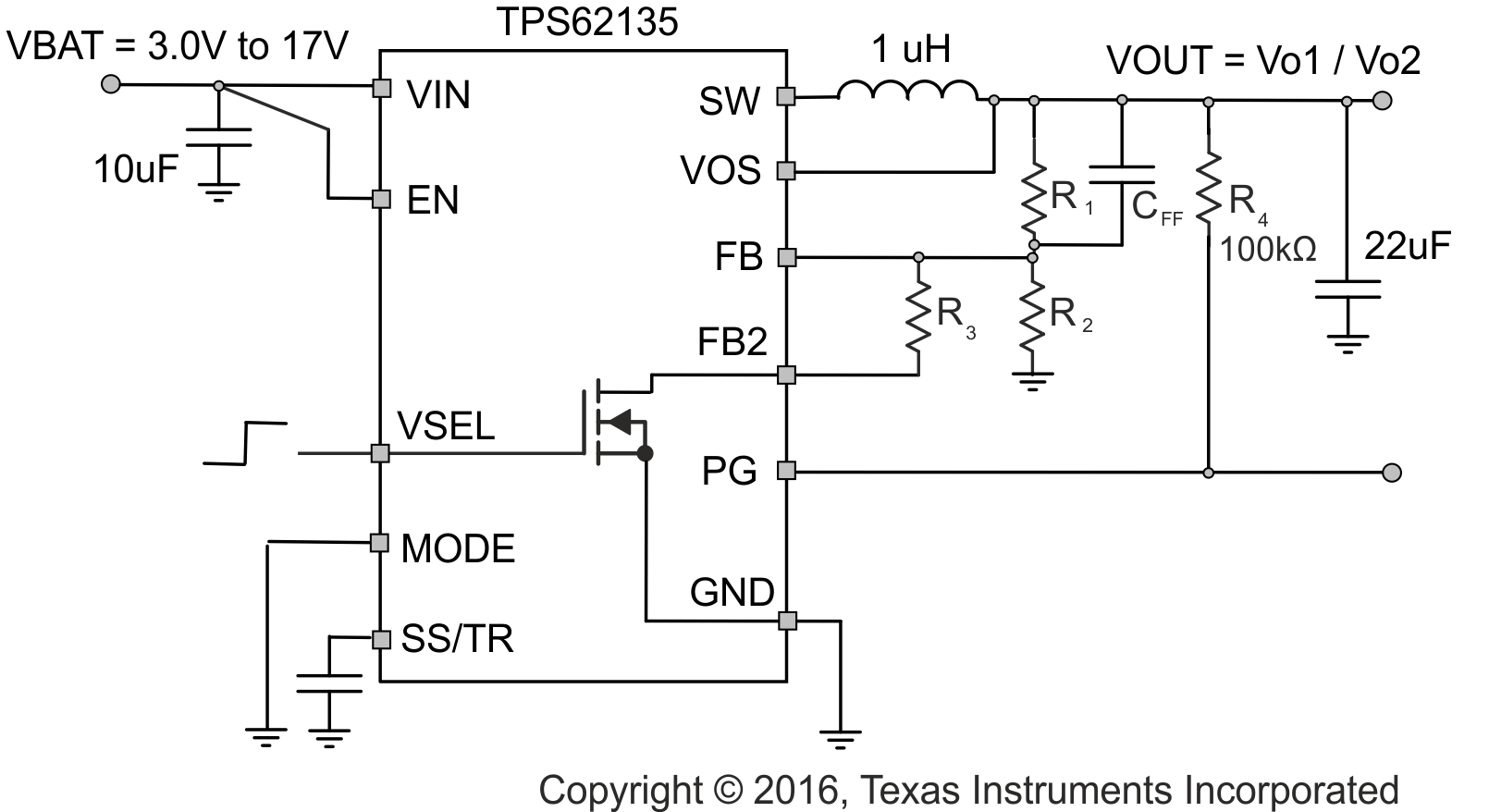Figure 83. Typical Application using VSEL

#### 10.2.2.1 Design Requirements

VSEL allows to switch between two output voltages by changing the output voltage divider ratio. This is done by an internal MOSFET connecting resistor R3 to GND. Pulling VSEL high turns on the MOSFET that connects R3 in parallel to R2. The divider ratio is changed such that the output voltage increases from Vo1 to Vo2.

When the output voltage is ramped down and the device is in forced PWM mode, the device will sink current.

#### 10.2.2.2 Detailed Design Procedure

TPS62135x typically does not require a feed forward capacitor in parallel to R1. For a large voltage change such as 3.3 V to 5 V, a small feed forward capacitor CFFhelps to improve the settling behavior. In order to switch from an output voltage of for example 3.3 V to an output voltage of 5 V, set the resistor divider for R1 and R2 to 3.3V and calculate R3 with Equation 17. With R1 = 560 kΩ and R2 = 150 kΩ this gives R3 = 232 kΩ. A feedforward capacitor of 12 pF was used to get a voltage transition as shown below.

Equation 17.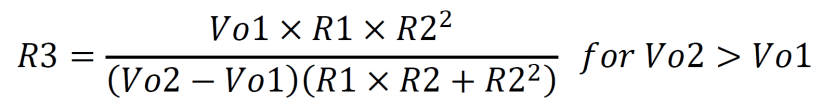#### 10.2.2.3 Application Curves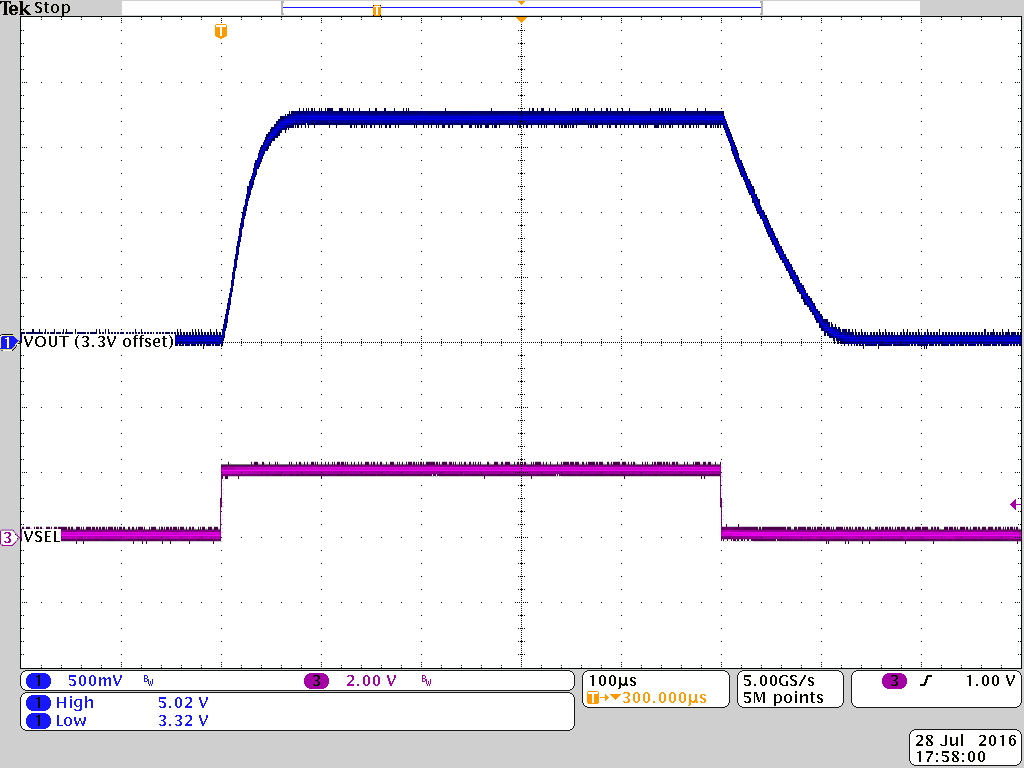Figure 84. Output Voltage Change from 3.3 V to 5 V in PFM with 20 Ω load resistance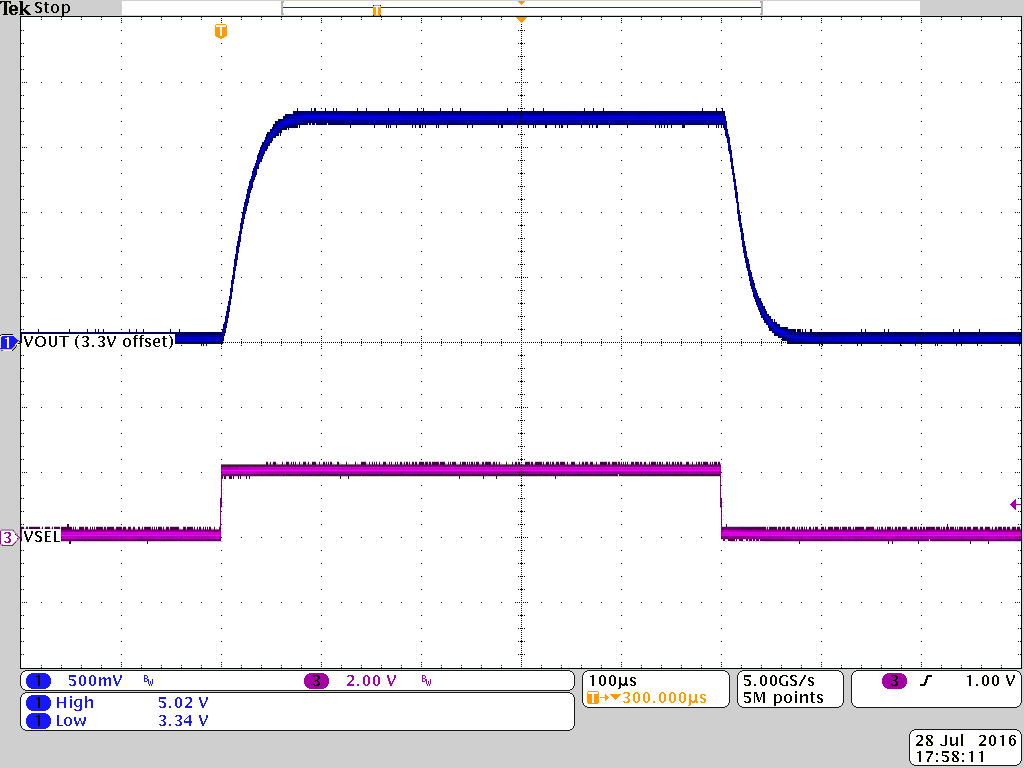Figure 85. Output Voltage Change from 3.3 V to 5 V in PWM with 20 Ω load resistance

### 10.3 System Examples

#### 10.3.1 LED Power Supply

The TPS62135x can be used as a power supply for power LEDs. The FB pin can be easily set down to lower values than nominal by using the SS/TR pin. With that, the voltage drop on the sense resistor is low to avoid excessive power loss. Since this pin provides 2.5 µA, the feedback pin voltage can be adjusted by an external resistor per Equation 18. This drop, proportional to the LED current, is used to regulate the output voltage (anode voltage) to a proper level to drive the LED. Both analog and PWM dimming are supported with the TPS62135x. Figure 86 shows an application circuit, tested with analog dimming: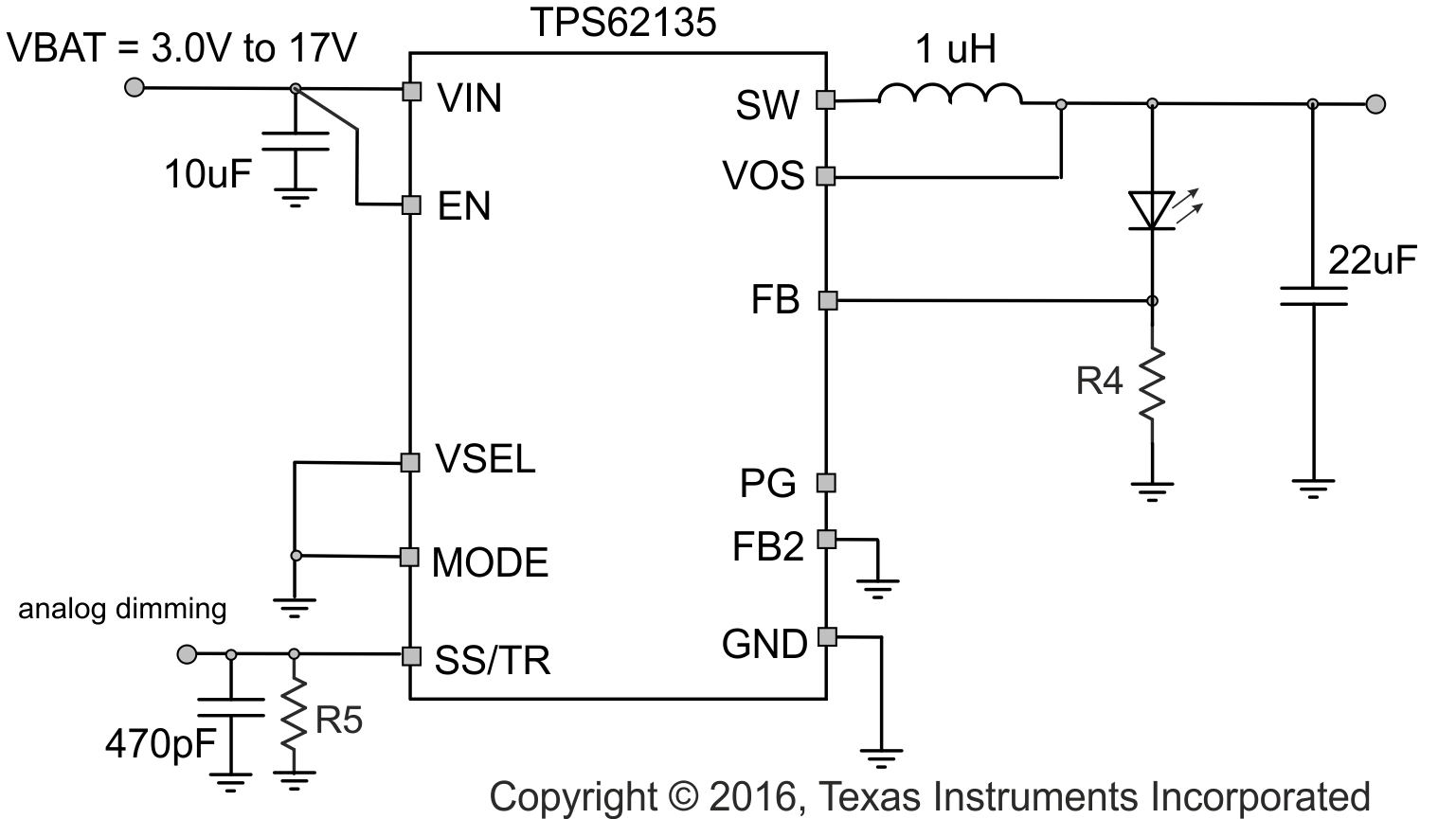Figure 86. Single Power LED Supply

spacing

The resistor at SS/TR defines the FB voltage. It is set to 350 mV by R5 = 140 kΩ using Equation 18. This cuts the losses on R4 to half from the nominal 0.7 V of feedback voltage while it still provides good accuracy.

spacing

Equation 18.spacing

The device now supplies a constant current set by resistor R4 from FB to GND. The minimum input voltage has to be rated according the forward voltage needed by the LED used. More information is available in the Application Note SLVA451.

spacing

In applications where TPS62135x is used to power multiple load circuits, it may be the case that the total capacitance on the output is very large. In order to properly regulate the output voltage, there needs to be an appropriate AC signal level on the VOS pin. Tantalum capacitors have a large enough ESR to keep output voltage ripple sufficiently high on the VOS pin. With low ESR ceramic capacitors, the output voltage ripple may get very low, so it is not recommended to use a large capacitance directly on the output of the device. If there are several load circuits with their associated input capacitor on a pcb, these loads are typically distributed across the board. This adds enough trace resistance (Rtrace) to keep a large enough AC signal on the VOS pin for proper regulation.

The minimum total trace resistance on the distributed load is 10 mΩ. The total capacitance n x Cin in the use case below was 32 x 47 uF of ceramic X7R capacitors.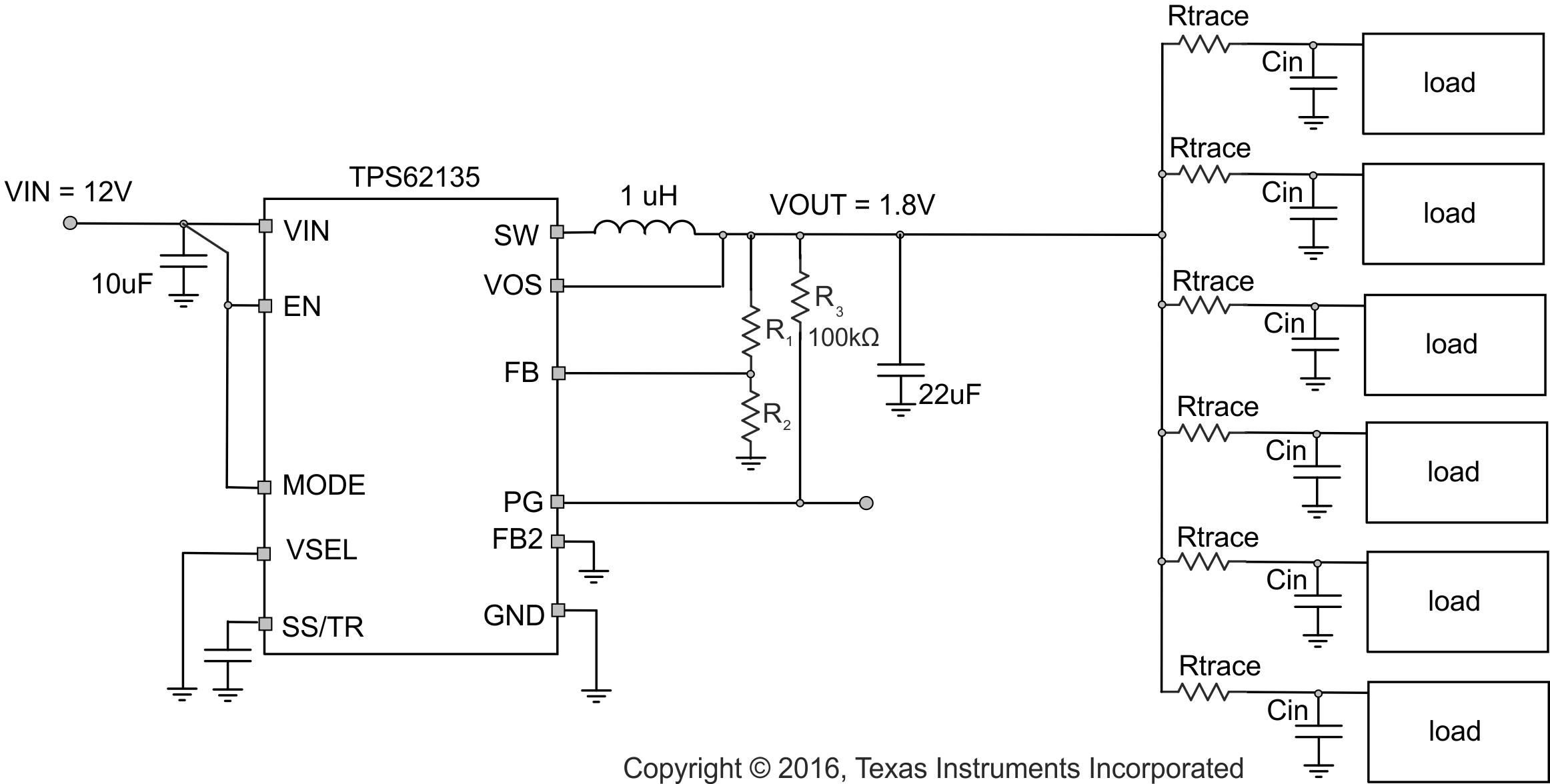Figure 87. Multiple Loads

#### 10.3.3 Voltage Tracking

DEVICE 2 follows the voltage applied to the SS/TR pin. A ramp on SS/TR to 0.7 V ramps the output voltage according to the 0.7 V reference.

Tracking the 3.3 V of DEVICE 1 requires a resistor divider on SS/TR of DEVICE 2 equal to the output voltage divider of DEVICE 1. The output current of 2.5µA from the SS/TR pin cases an offset voltage on the resistor divider formed by R5 and R6. The equivalent resistance of R6 // R5 should therefore be kept below 15kΩ.Figure 88. Tracking Example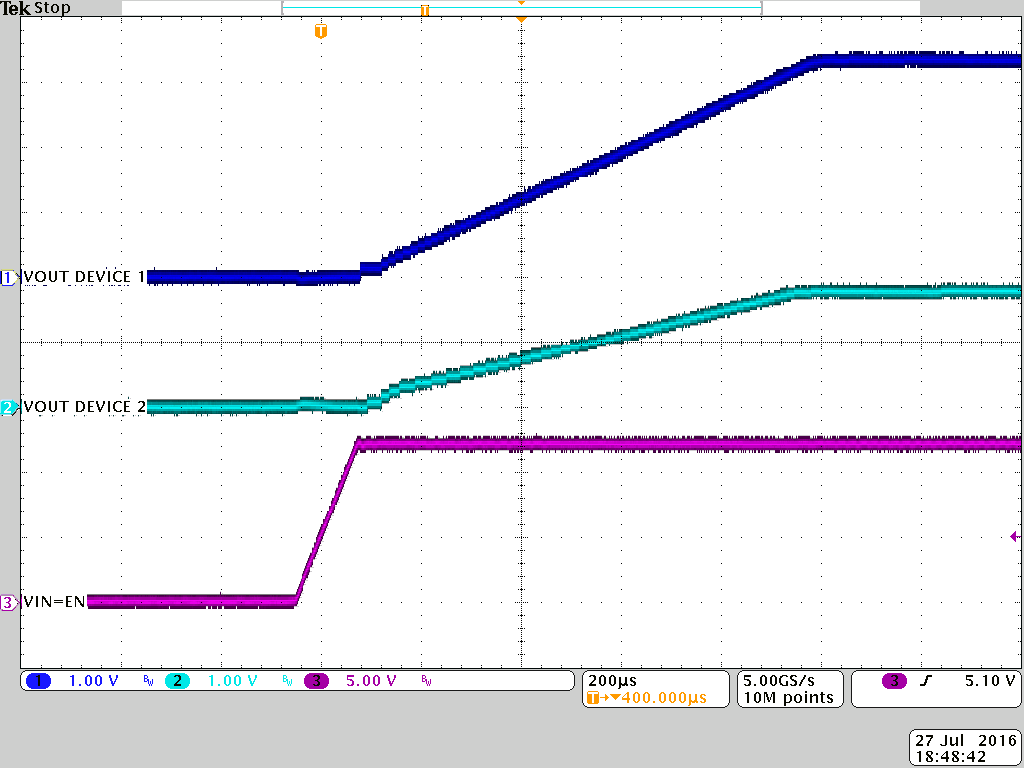Figure 89. Tracking

#### 10.3.4 Precise Soft-Start Timing

The SS/TR pin of the TPS62135x can be used for tracking as well as for setting the Soft-Start time. The TPS62135x has one GND terminal which is used for the power ground as well as for the analog ground connection. While starting the device with a load current above approximately 1 A, the noise on the GND connection can lead to a Soft-Start time shorter than calculated. There are two external work arounds as given below.

Adding a 10 kΩ resistor filters the noise on the GND connection and keeps the Soft-Start time at the value calculated.

Figure 91 does not require an external component. It provides a connection to the internal analog ground by using the FB2 pin and its internal NMOS to that node. The internal NMOS needs to be turned ON by setting VSEL = high.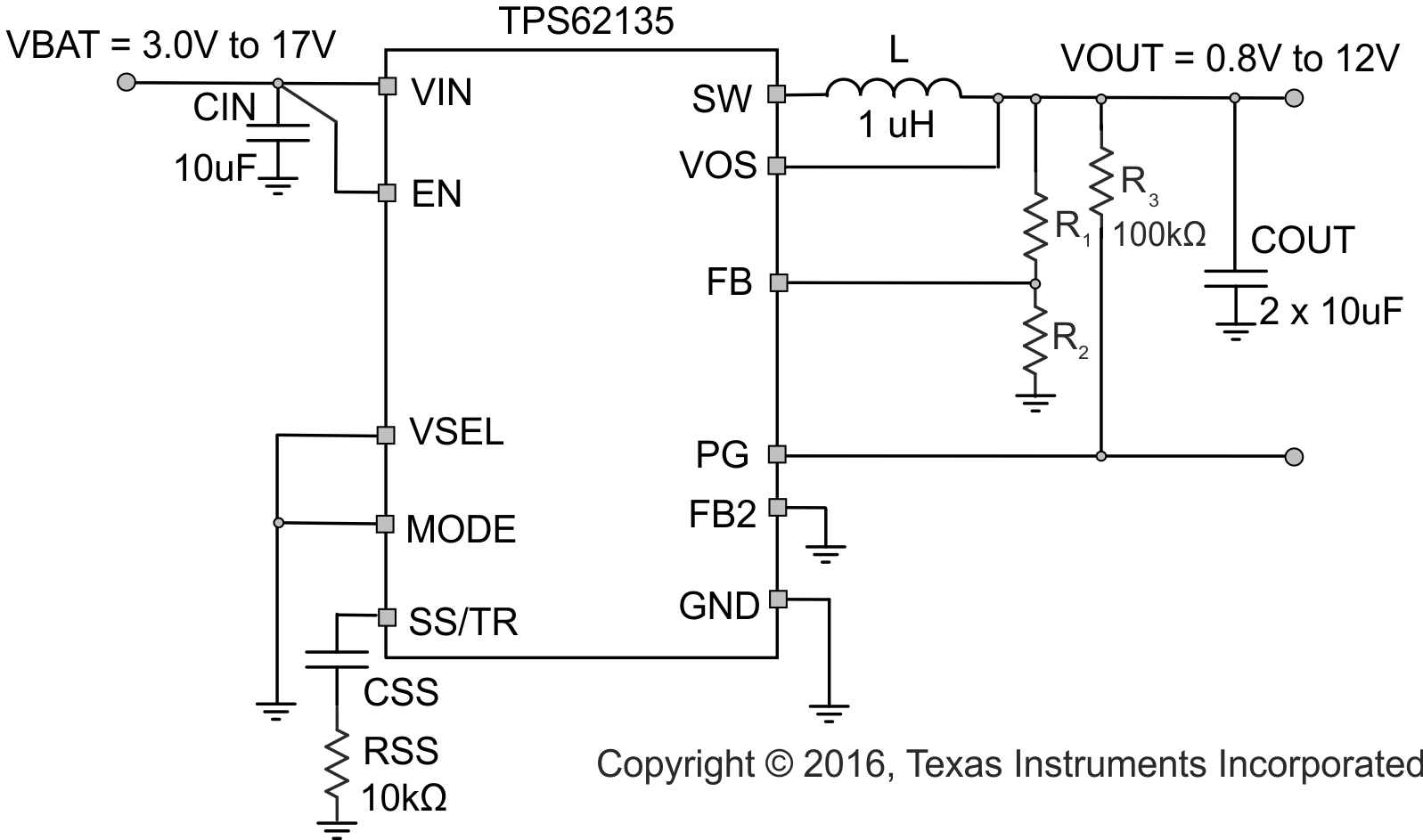Figure 90. Adding a Series Resistor to CSS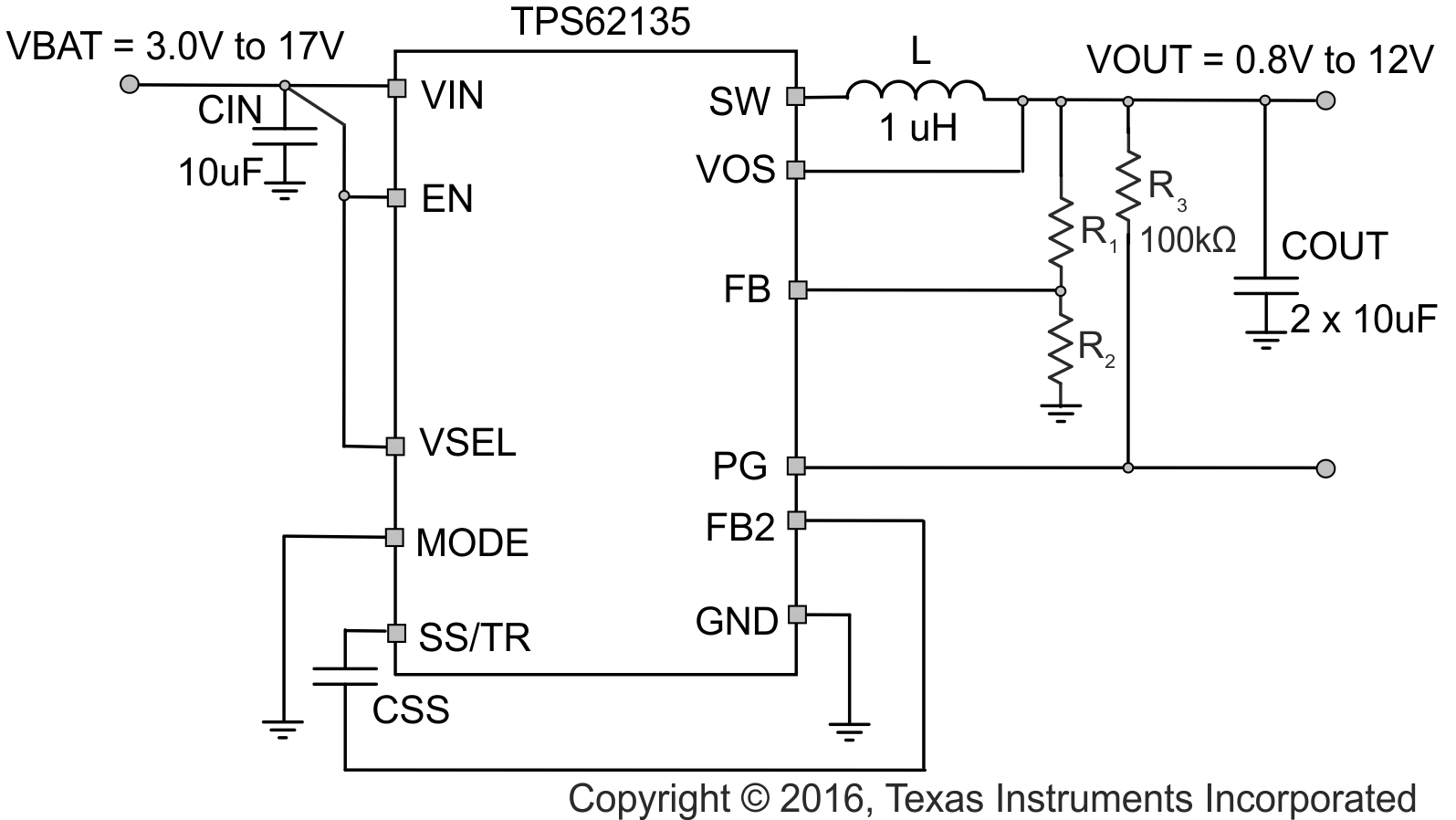Figure 91. Connecting CSS to the Internal Analog Ground by using FB2